Win up to 100% scholarship on Aakash BYJU'S JEE/NEET courses with ABNAT Win up to 100% scholarship on Aakash BYJU'S JEE/NEET courses with ABNAT

# JEE Advanced Question Paper 2022 Physics Paper 1

## JEE Advanced 2022 Physics Question Paper 1 with Solutions

SECTION – 1 (Maximum marks : 24)

∙ This section contains EIGHT (08) questions.

∙ The answer to each question is a NUMERICAL VALUE.

∙ For each question, enter the correct numerical value of the answer using the mouse and the on-screen virtual numeric keypad in the place designated to enter the answer. If the numerical value has more than two decimal places, truncate/round-off the value to TWO decimal places.

∙ Answer to each question will be evaluated according to the following marking scheme:

Full Marks : +3 ONLY if the correct numerical value is entered;

Zero Marks : 0 In all other cases.

1. Two spherical stars A and B have densities ρA and ρB, respectively. A and B have the same radius, and their masses MA and MB are related by MB = 2MA. Due to an interaction process, star A loses some of its mass, so that its radius is halved, while its spherical shape is retained, and its density remains ρA. The entire mass lost by A is deposited as a thick spherical shell on B with the density of the shell being ρA. If vA and vB are the escape velocities from A and B after the interaction process, the ratio

$$\begin{array}{l}\frac{{{v_B}}}{{{v_A}}} = \sqrt {\frac{{10n}}{{{{15}^{1/3}}}}} .\end{array}$$
The value of n is ______.

Sol.

$$\begin{array}{l}{v_A} = \sqrt {\frac{{2G{M_A}}}{{8 \times \left( {\frac{R}{2}} \right)}}}=\frac{{{v_0}}}{2}\end{array}$$

For B,

$$\begin{array}{l}\frac{4}{3}\pi \left( {{r^3} – {R^3}} \right) = \frac{4}{3}\pi {R^3} \times \frac{7}{8}\end{array}$$
$$\begin{array}{l}\Rightarrow r = {\left( {\frac{{15}}{8}} \right)^{\frac{1}{3}}}R\end{array}$$
$$\begin{array}{l}\therefore \sqrt{\frac{2G\times \left ( 2M_A+\frac{7}{8}M_A \right )}{\frac{(15)^\frac{1}{3}R}{2}}} \end{array}$$
$$\begin{array}{l}=v_0\times \sqrt{\frac{23\times 2}{8\times (15)^\frac{1}{3}}} \end{array}$$
$$\begin{array}{l}\therefore \frac{v_B}{v_A}=\sqrt{\frac{23}{(15)^\frac{1}{3}}}=\sqrt{\frac{2.30\times 10}{(15)^\frac{1}{3}}}\end{array}$$

2. The minimum kinetic energy needed by an alpha particle to cause the nuclear reaction

$$\begin{array}{l}_7^{16}N + \,\,_2^4He \to \,_1^1H + \,_8^{19}O\end{array}$$
in a laboratory frame is n (in MeV). Assume that
$$\begin{array}{l}_7^{16}N\ \text{is at rest in the laboratory frame.}\end{array}$$

$$\begin{array}{l}\text{The masses of}\ _7^{16}N,\,\,_2^4He,\,\,_1^1H\ \text{and}\ _8^{19}O\end{array}$$
can be taken to be 16.006 u, 4.003 u, 1.008 u and 19.003 u, respectively, where 1 u = 930 MeVc–2. The value of n is _______.

Sol. Q = (mN + mHe – mH – mO) × c2

= (16.006 + 4.003 – 1.008 – 19.003) × 930 MeV

= – 1.86 MeV

= 1.86 MeV energy absorbed.

And,

$$\begin{array}{l}\frac{1}{2} \times \frac{{m \times 4m}}{{5m}} \times {v^2} = {\text{max loss in kinetic energy}}\end{array}$$
$$\begin{array}{l}\Rightarrow \frac{1}{2}m{v^2} = \frac{5}{4}\; \times Q\end{array}$$
$$\begin{array}{l}= \frac{5}{4} \times (1.86)\;{\rm{MeV}}\\ = 2.325 MeV\\ \therefore n = 2.33\end{array}$$

3. In the following circuit C1 = 12 μF, C2 = C3 = 4 μF and C4 = C5 = 2 μF. The charge stored in C3 is ____ μC.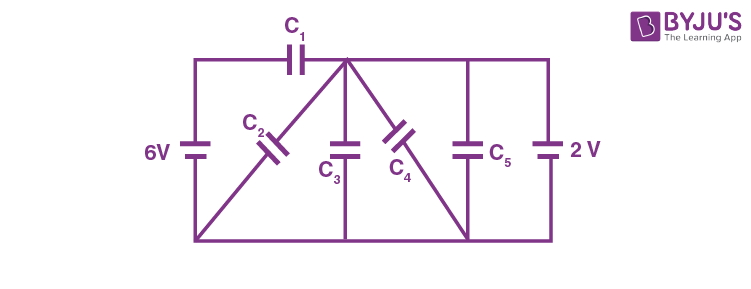Sol. From circuit given,

Potential difference across C3 is 2 V (constant)

Q3 = 2 × 4 μC

= 8 μC

4. A rod of length 2 cm makes an angle 2π/3 rad with the principal axis of a thin convex lens. The lens has a focal length of 10 cm and is placed at a distance of 40/3 cm from the object as shown in the figure. The height of the image is (30√3)/13 cm and the angle made by it with respect to the principal axis is α rad. The value of α is π/n rad, where n is _______.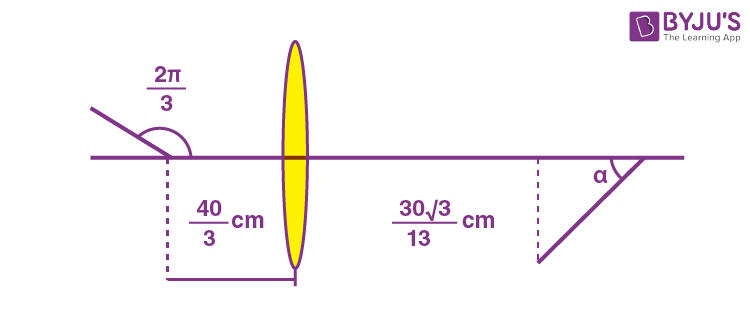Sol.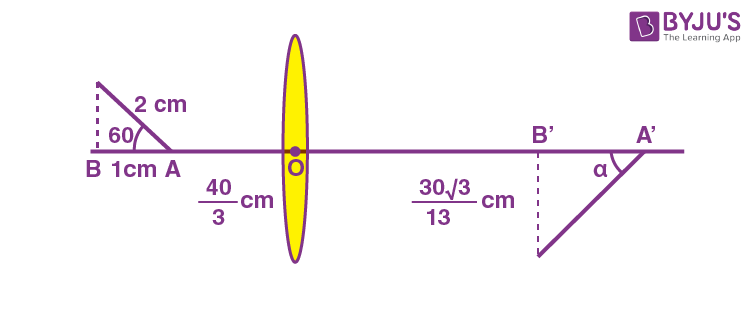$$\begin{array}{l}OA’= \frac{\frac{40}{3}\times 10}{\frac{43}{3} – 10} = 40\ cm\end{array}$$
$$\begin{array}{l}OB’= \frac{\frac{43}{3}\times 10}{\frac{43}{3} – 10} = \frac{430}{13}\ cm\end{array}$$
$$\begin{array}{l}\therefore A’B’ = 40-\frac{430}{13} = \frac{90}{13}\ cm\end{array}$$
$$\begin{array}{l} \therefore \tan \alpha=\frac{30\sqrt 3}{13\times \left(\frac{90}{13}\right)} = \frac{1}{\sqrt{3}}\end{array}$$
$$\begin{array}{l}\Rightarrow \alpha = \frac{\pi }{6}\end{array}$$

∴ n = 6.00

5. At time t = 0, a disk of radius 1 m starts to roll without slipping on a horizontal plane with an angular acceleration of α = ⅔ rad s-2. A small stone is stuck to the disk. At t = 0, it is at the contact point of the disk and the plane. Later, at time t = √π s the stone detaches itself and flies off tangentially from the disk. The maximum height (in m) reached by the stone measured from the plane is

$$\begin{array}{l}\frac{1}{2} + \frac{x}{{10}}.\end{array}$$
The value of x is _____. [Take g = 10 ms–2.]

Sol. The angle rotated by disc in t = √π s is

$$\begin{array}{l}\theta={\omega _0}t + \frac{1}{2}\alpha{t^2}\end{array}$$
$$\begin{array}{l}\Rightarrow \theta=\frac{1}{2}\times \frac{2}{3}\left(\sqrt\pi\right)^2\end{array}$$
$$\begin{array}{l} = \frac{\pi }{3}\ rad\end{array}$$

and the angular velocity of disc is

ω = ω0 + αt

$$\begin{array}{l} = \frac{2\sqrt \pi}{3}{\rm{rad/s}}\end{array}$$

and

$$\begin{array}{l}v_{cm} = \omega R = \frac{2\sqrt \pi}{3} \times 1\end{array}$$
$$\begin{array}{l} = \frac{2\sqrt\pi}{3}{m/s}\end{array}$$

So, at the moment it detaches the situation is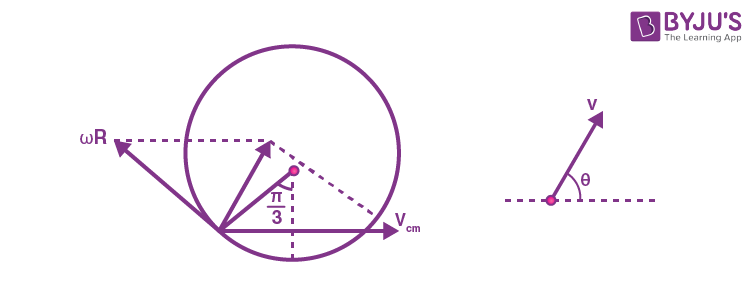$$\begin{array}{l}v = \sqrt {{{\left( {\omega R} \right)}^2} + v_{{\rm{cm}}}^2 + 2\left( {\omega R} \right){v_{{\rm{cm}}}}\cos 120^\circ } \end{array}$$
$$\begin{array}{l} = v_{cm} = \frac{2\sqrt \pi}{3}{m/s}\end{array}$$

and

$$\begin{array}{l}\tan\theta=\frac{\omega R \sin 120^\circ}{v_{cm}+\omega R \cos 120^\circ}\end{array}$$
$$\begin{array}{l}\Rightarrow \tan \theta=\sqrt 3\end{array}$$
$$\begin{array}{l} \Rightarrow \theta=\frac{\pi}{3}\ rad\end{array}$$

So,

$$\begin{array}{l}{H_{\max }} = \frac{{{u^2}{{\sin }^2}\theta }}{{2g}}\end{array}$$
$$\begin{array}{l} =\frac{\left( \frac{2\sqrt{\pi}}{3}\right)^2\times \sin^260^\circ}{2\times 10}\end{array}$$
$$\begin{array}{l} = \frac{{4\pi\times 3}}{{9 \times 2 \times 10 \times 4}}\end{array}$$
$$\begin{array}{l} = \frac{\pi}{60}\ m\end{array}$$

So, height from ground will be

$$\begin{array}{l}R\left( {1 – \cos 60^\circ } \right) + \frac{\pi }{{60}} = \frac{1}{2} + \frac{x}{{10}}\end{array}$$
$$\begin{array}{l}\Rightarrow x = \frac{\pi }{6} = 0.52\end{array}$$

6. A solid sphere of mass 1 kg and radius 1 m rolls without slipping on a fixed inclined plane with an angle of inclination θ = 30° from the horizontal. Two forces of magnitude 1 N each, parallel to the incline, act on the sphere, both at distance r = 0.5 m from the center of the sphere, as shown in the figure. The acceleration of the sphere down the plane is ______ ms–2. (Take g = 10 ms–2.)Sol.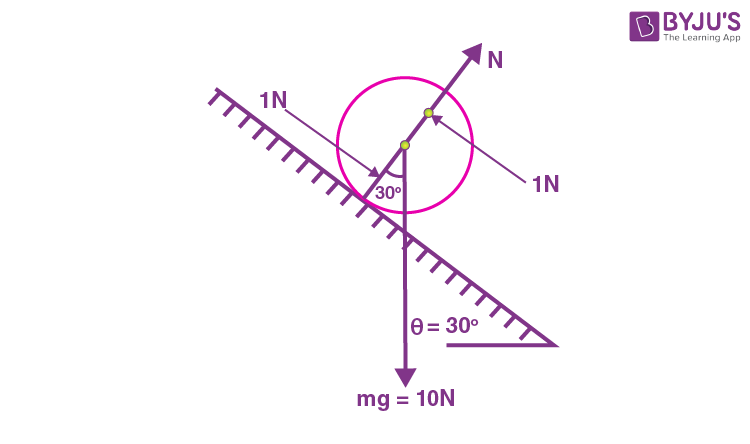$$\begin{array}{l}\vec \tau \; = \;mgR\;\sin \;{30^ \otimes } + \;1\; \times \;{1^ \odot }\end{array}$$
$$\begin{array}{l}\text{taking}\ \otimes\ \text{is positive}\end{array}$$
$$\begin{array}{l}5 – 1=\frac{7}{5}\;m{R^2}\alpha \end{array}$$
$$\begin{array}{l}\Rightarrow \alpha=\frac{20}{7}\ rad/s^2\end{array}$$

So,

$$\begin{array}{l}a_{cm}=\alpha R=\frac{20}{7}\ m/s^2\end{array}$$
$$\begin{array}{l}a_{cm} = 2.86 ~\text{m/s}^2\end{array}$$

7. Consider an LC circuit, with inductance L = 0.1 H and capacitance C = 10–3 F, kept on a plane. The area of the circuit is 1 m2. It is placed in a constant magnetic field of strength B0, which is perpendicular to the plane of the circuit. At time t = 0, the magnetic field strength starts increasing linearly as B = B0 + βt with β = 0.04 Ts–1. The maximum magnitude of the current in the circuit is _____ mA.

Sol. Emf induced in the circuit is

$$\begin{array}{l}\left| E \right| = \left| {\frac{{d\phi }}{{dt}}} \right| = \frac{d}{{dt}}\left( {\left( {{B_0} + \beta t} \right)A} \right)\end{array}$$

= β × A

= 0.04 V

So the circuit can be rearranged as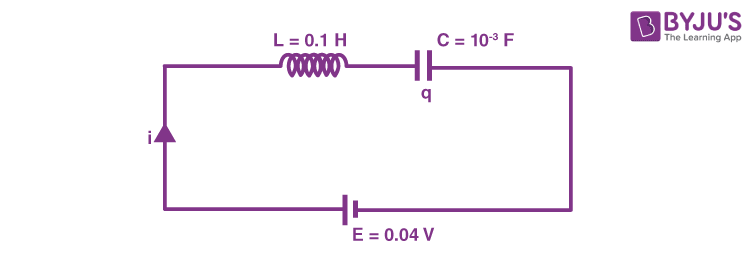Using Kirchhoff’s law we can write

$$\begin{array}{l}E = L\frac{{di}}{{dt}} + \frac{q}{C}\end{array}$$
$$\begin{array}{l}L\frac{{di}}{{dt}} = E – \frac{q}{C}\end{array}$$

Or

$$\begin{array}{l}\frac{d^2q}{dt^2}=-\frac{1}{LC}(q – CE)\end{array}$$

Using the SHM concept, we can write

q = CE + A sin(ωt + φ)

$$\begin{array}{l}\left( where\ \omega =\frac{1}{\sqrt{LC}}\right)\end{array}$$

at t = 0, q = 0 & i = 0

So A = CE &

$$\begin{array}{l}\phi = – \frac{\pi}{2}\end{array}$$

q = CECE cos ωt

So

$$\begin{array}{l}i = \frac{{dq}}{{dt}} = CE\omega \,\,\sin \omega t\end{array}$$

So

$$\begin{array}{l}{i_{\max }} = \frac{{{{10}^{ – 3}} \times 0.04}}{{\sqrt {0.1 \times {{10}^{ – 3}}} }}\\= 4 mA\end{array}$$

8. A projectile is fired from horizontal ground with speed v and projection angle θ. When the acceleration due to gravity is g, the range of the projectile is d. If at the highest point in its trajectory, the projectile enters a different region where the effective acceleration due to gravity is g’= g/0.81, then the new range is d′ = nd. The value of n is _____.

Sol.

$$\begin{array}{l}d = \frac{{{u^2}\sin 2\theta }}{g}\end{array}$$
$$\begin{array}{l}H = \frac{{{u^2}{{\sin }^2}\theta }}{{2g}}\end{array}$$

So, after entering in the new region, time taken by projectile to reach ground

$$\begin{array}{l}t = \sqrt {\frac{{2H}}{{g^\prime }}} \end{array}$$
$$\begin{array}{l} = \sqrt{\frac{2u^2\sin^2\theta\times 0.81}{2g \times g}} \end{array}$$
$$\begin{array}{l} = \frac{{0.94\sin \theta }}{g}\end{array}$$

So, horizontal displacement done by the projectile in new region is

$$\begin{array}{l}x = \frac{{0.9u\sin \theta }}{g} \times u\cos \theta \end{array}$$
$$\begin{array}{l} = 0.9\frac{{{u^2}\sin 2\theta }}{{2g}}\end{array}$$

So,

$$\begin{array}{l}d^\prime = \frac{d}{2} + x= 0.95d\end{array}$$

So, n = 0.95d

SECTION – 2 (Maximum marks : 24)

This section contains SIX (06) questions.

Each question has FOUR options (A), (B), (C) and (D). ONE OR MORE THAN ONE of these four option(s) is(are) correct answer(s).

∙ For each question, choose the option(s) corresponding to (all) the correct answer(s).

∙ Answer to each question will be evaluated according to the following marking scheme:

Full Marks : +4 ONLY if (all) the correct option(s) is(are) chosen;

Partial Marks : +3 If all the four options are correct but ONLY three options are chosen;

Partial Marks : + 2 If three or more options are correct but ONLY two options are chosen, both of which are correct;

Partial Marks : +1 If two or more options are correct but ONLY one option is chosen and it is a correct option;

Zero Marks : 0 If none of the options is chosen (i.e. the question is unanswered);

Negative Marks : –2 In all other cases.

9. A medium having dielectric constant K >1 fills the space between the plates of a parallel plate capacitor. The plates have large area, and the distance between them is d. The capacitor is connected to a battery of voltage V, as shown in Figure (a). Now, both the plates are moved by a distance of d/2 from their original positions, as shown in Figure (b).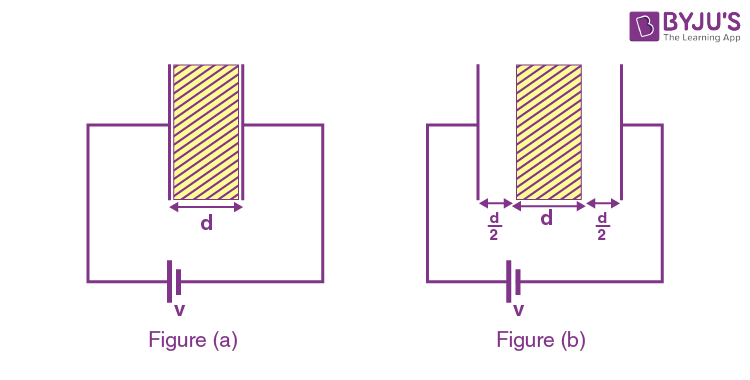In the process of going from the configuration depicted in Figure (a) to that in Figure (b), which of the following statement(s) is(are) correct?

(A) The electric field inside the dielectric material is reduced by a factor of 2K.

(B) The capacitance is decreased by a factor of 1/(K + 1).

(C) The voltage between the capacitor plates is increased by a factor of (K + 1).

(D) The work done in the process DOES NOT depend on the presence of the dielectric material

Sol.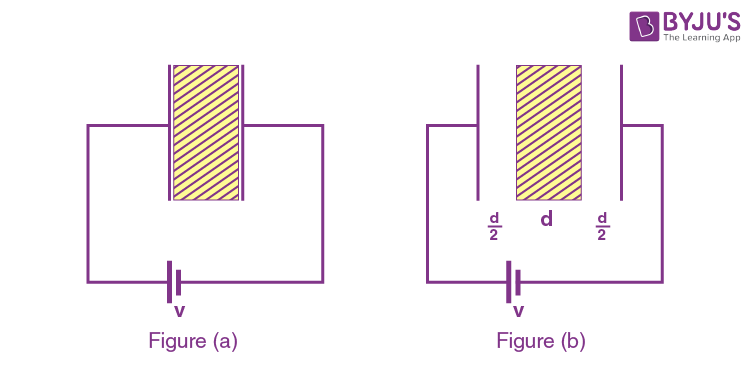$$\begin{array}{l}{C_a} = \frac{{K{\varepsilon _0}A}}{d}\end{array}$$
$$\begin{array}{l}{q_a} = \frac{{K{\varepsilon _0}A}}{d}V\end{array}$$
$$\begin{array}{l}{E_a} = \frac{{{q_a}}}{{KA{\varepsilon _0}}} = \frac{{K{\varepsilon _0}AV}}{{dK{\varepsilon _0}A}}\end{array}$$
$$\begin{array}{l} = \frac{V}{d}\end{array}$$
$$\begin{array}{l}{C_b} = \frac{{{\varepsilon _0}A}}{{d + \left( {\frac{d}{K}} \right)}}\end{array}$$
$$\begin{array}{l} = \frac{{{\varepsilon _0}AK}}{{d\left( {K + 1} \right)}}\end{array}$$
$$\begin{array}{l}{q_b} = \frac{{{\varepsilon _0}AKV}}{{d\left( {K + 1} \right)}}\end{array}$$
$$\begin{array}{l}(E_b)_{\text{dielectric}} = \frac{E_{air}}{K}\end{array}$$
$$\begin{array}{l} = \frac{{{q_b}}}{{KA{\varepsilon _0}}}\end{array}$$
$$\begin{array}{l} = \frac{{{\varepsilon _0}AKV}}{{d\left( {K + 1} \right)\left( {KA{\varepsilon _0}} \right)}}\end{array}$$
$$\begin{array}{l} = \frac{V}{{d\left( {K + 1} \right)}}\end{array}$$

Capacitance decreases by a factor of 1/(K + 1)

Work done in the process = UfUi

$$\begin{array}{l} = \frac{1}{2}\left( {{C_f} – {C_i}} \right){V^2}\end{array}$$
$$\begin{array}{l} = \frac{1}{2}\left( {\frac{{{\varepsilon _0}AK}}{{d\left( {K + 1} \right)}} – \frac{{K{\varepsilon _0}A}}{d}} \right){V^2}\end{array}$$
$$\begin{array}{l} = \frac{1}{2}{V^2}\frac{{{\varepsilon _0}AK}}{d}\left( {\frac{1}{{K + 1}} – 1} \right)\end{array}$$
$$\begin{array}{l} = \frac{1}{2}\frac{{{\varepsilon _0}AK{V^2}}}{d}\frac{{1 – K – 1}}{{K + 1}}\end{array}$$
$$\begin{array}{l} = \frac{1}{2}\frac{{{\varepsilon _0}A{V^2}}}{d}\left( {\frac{{ – {K^2}}}{{K + 1}}} \right)\end{array}$$

10. The figure shows a circuit having eight resistances of 1 Ω each, labelled R1 to R8, and two ideal batteries with voltages ε1 = 12 V and ε2 = 6 V.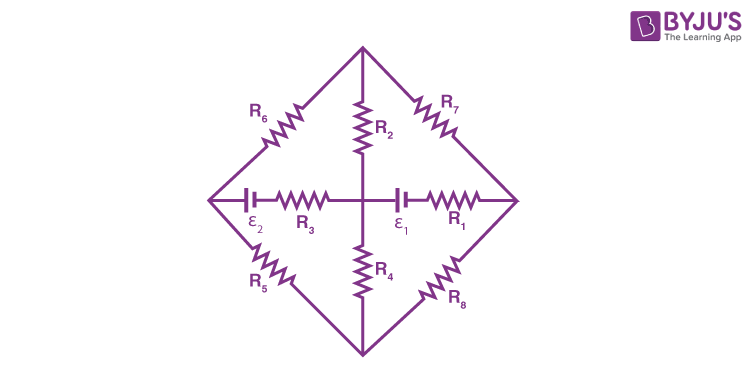Which of the following statement(s) is(are) correct?

(A) The magnitude of current flowing through R1 is 7.2 A.

(B) The magnitude of current flowing through R2 is 1.2 A.

(C) The magnitude of current flowing through R3 is 4.8 A.

(D) The magnitude of current flowing through R5 is 2.4 A.

Sol.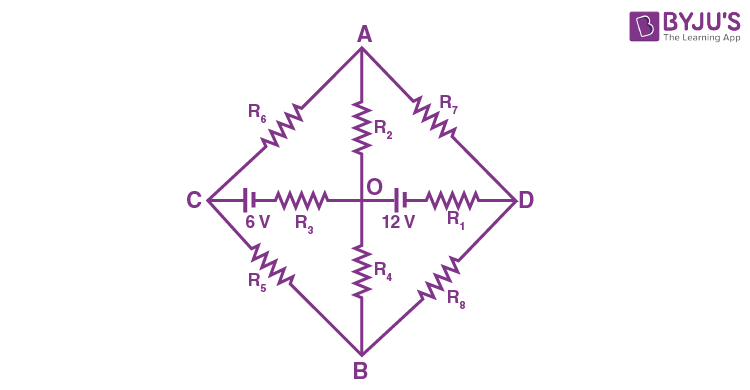Point A and B are at same potential so they can be merged/folded.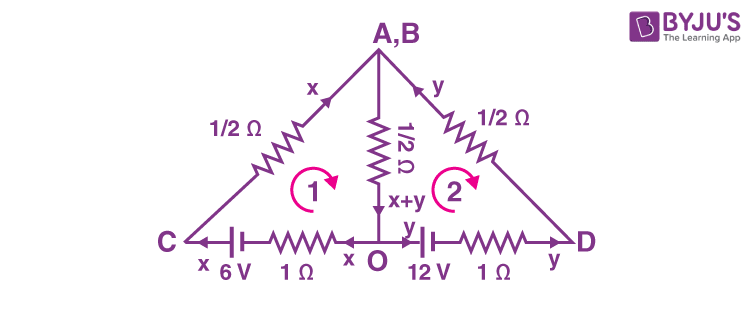For loop 1

$$\begin{array}{l} – \frac{1}{2}x – \frac{1}{2}(x + y) – x + 6 = 0\end{array}$$
$$\begin{array}{l} -2x-\frac{y}{2}=-6\end{array}$$

–4xy = –12

4x + y = 12 …(1)

For loop 2

$$\begin{array}{l}\frac{1}{2}y + 1y + 12 + \frac{1}{2}(x + y) = 0\end{array}$$
$$\begin{array}{l}\frac{3}{2}y + \frac{y}{2} + \frac{x}{2} = – 12\end{array}$$
$$\begin{array}{l}2y + \frac{x}{2} =- 12\end{array}$$

4y + x = –24 …(2)

4y + x – 16x – 4y

= –24 – 48

–15x = –72

$$\begin{array}{l}x = \frac{72}{15}\end{array}$$
$$\begin{array}{l}4\left( {\frac{{72}}{{15}}} \right) + y = 12\end{array}$$
$$\begin{array}{l}y = 12 – \frac{{288}}{{15}}\end{array}$$
$$\begin{array}{l} = \frac{180 – 288}{15}\end{array}$$
$$\begin{array}{l} = \frac{-108}{15}=-7.2\ A\end{array}$$

Current in R1 = 7.2 A

$$\begin{array}{l}\text{Current in }\ {R_2} = \frac{{x + y}}{2} = \left( {\frac{{72}}{{15}} – \frac{{108}}{{15}}} \right)\frac{1}{2}\end{array}$$
$$\begin{array}{l} = \frac{1}{2}\left( {\frac{{36}}{{15}}} \right) = \frac{{2.4}}{2}\;{\rm{A}}\end{array}$$

= 1.2 A

Current in R3 = x = 4.8 A

Current in

$$\begin{array}{l}{R_5} = \frac{1}{2}x = 2.4\;{\rm{A}}\end{array}$$

11. An ideal gas of density ρ = 0.2 kg m–3 enters a chimney of height h at the rate of α = 0.8 kg s–1 from its lower end, and escapes through the upper end as shown in the figure. The cross-sectional area of the lower end is A1 = 0.1 m2 and the upper end is A2 = 0.4 m2. The pressure and the temperature of the gas at the lower end are 600 Pa and 300 K, respectively, while its temperature at the upper end is 150 K. The chimney is heat insulated so that the gas undergoes adiabatic expansion. Take g = 10 ms–2 and the ratio of specific heats of the gas γ = 2. Ignore atmospheric pressure.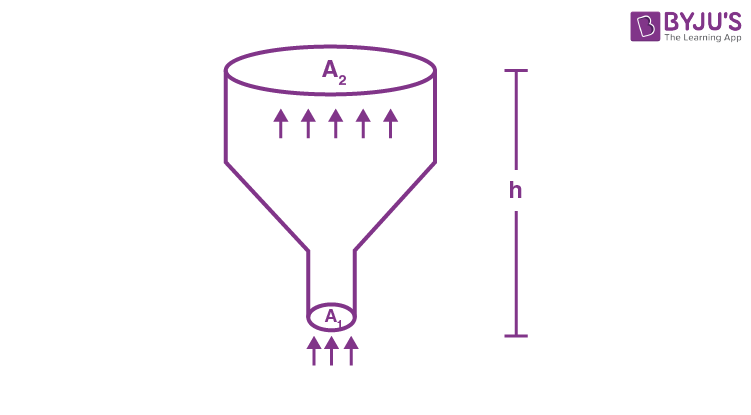Which of the following statement(s) is(are) correct?

(A) The pressure of the gas at the upper end of the chimney is 300 Pa.

(B) The velocity of the gas at the lower end of the chimney is 40 ms–1 and at the upper end is 20 ms–1.

(C) The height of the chimney is 590 m.

(D) The density of the gas at the upper end is 0.05 kg m–3 .

Sol.

$$\begin{array}{l}\frac{\rho _1}{\rho _2} = \left ( \frac{T_1}{T_2} \right )^\frac{1}{\gamma-1}\end{array}$$
$$\begin{array}{l}\Rightarrow {\rho _2} = \left( {0.2} \right){\left( {\frac{{150}}{{300}}} \right)^{\frac{1}{1}}} = 0.1{\rm{ kg/}}{{\rm{m}}^3}\end{array}$$
Rate of mass flow = 0.8 kg/s

⇒ Volume flow rate at top = 8 m3/s

$$\begin{array}{l}\frac{{{P_2}}}{{{P_1}}} = {\left( {\frac{{{\rho _2}}}{{{\rho _1}}}} \right)^\gamma } \Rightarrow {P_2} = 600{\left( {\frac{1}{2}} \right)^\gamma }\end{array}$$

Velocity of gas at lower end

$$\begin{array}{l} = \frac{{{V_1}}}{{{A_1}}} = \frac{{0.8}}{{0.2 \times 0.1}} = 40{\rm{ m/s}}\end{array}$$

Velocity of gas at upper end

$$\begin{array}{l} = \frac{{0.8 \times 2}}{{0.2 \times 0.4}} = 20{\rm{ m/s}}\end{array}$$

By applying energy conservation

$$\begin{array}{l}{\frac{1}{2}{\rho _1}v_1^2 + {P_1}}={\frac{1}{2}{\rho _2}v_2^2 + {P_2} + {\rho _2}{\rm{ }}g{h_2}} \end{array}$$
$$\begin{array}{l}\Rightarrow {\frac{1}{2}\left( {0.2} \right)\left( {1600} \right) + 600} = {\frac{1}{2}\left( {0.1} \right)\left( {400} \right) + 150 + \left( {0.1} \right)\left( {10} \right){h_2}} \end{array}$$
$$\begin{array}{l}\Rightarrow h_2 = 590 ~\text{m}\end{array}$$

12. Three plane mirrors form an equilateral triangle with each side of length L. There is a small hole at a distance l > 0 from one of the corners as shown in the figure. A ray of light is passed through the hole at an angle θ and can only come out through the same hole. The cross section of the mirror configuration and the ray of light lie on the same plane.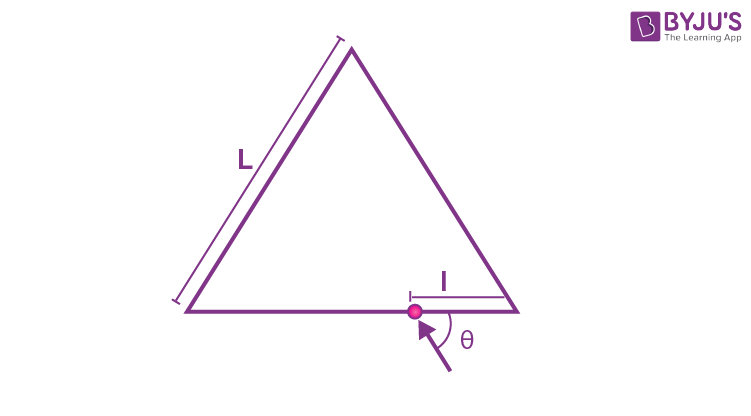Which of the following statement(s) is(are) correct?

(A) The ray of light will come out for θ = 30°, for 0 < 𝑙 < L.

(B) There is an angle for l = L/2 at which the ray of light will come out after two reflections.

(C) The ray of light will NEVER come out for θ = 60°, and l = L/3.

(D) The ray of light will come out for θ = 60°, and 0 < 𝑙 < L/2 after six reflections.

Sol.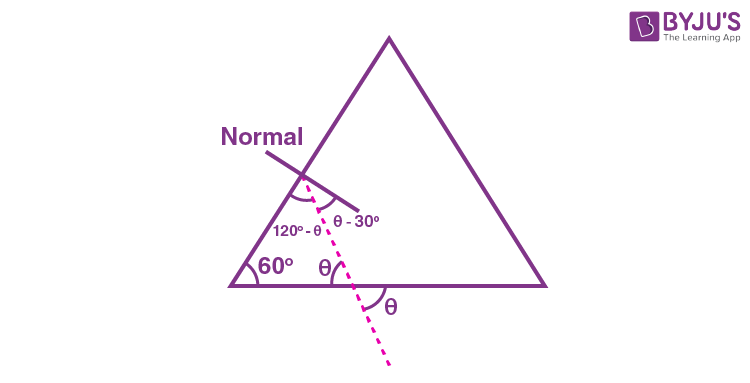As we can see, for θ = 30°, the ray will incident normally and hence will retrace its path.

⇒ (A) is correct

Considering the symmetry of the situation, we can have :⇒ (B) is correct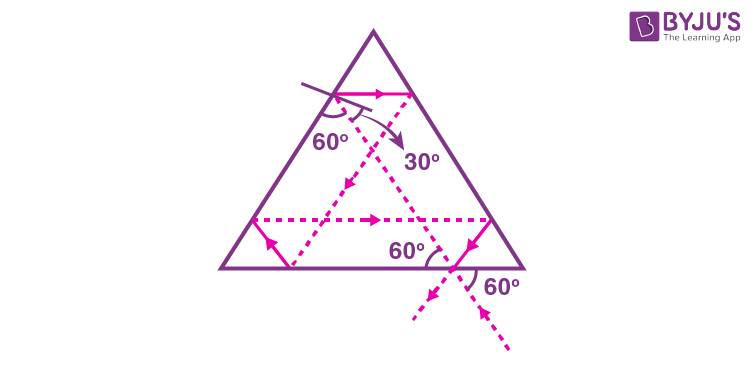As is clear from the above diagram, ray comes out.

⇒ (C) is not correct

Also, as is clear from the above diagram, total number of reflections = 5.

⇒ (D) is not correct.

13. Six charges are placed around a regular hexagon of side length 𝑎 as shown in the figure. Five of them have charge 𝑞, and the remaining one has charge x. The perpendicular from each charge to the nearest hexagon side passes through the center O of the hexagon and is bisected by the side.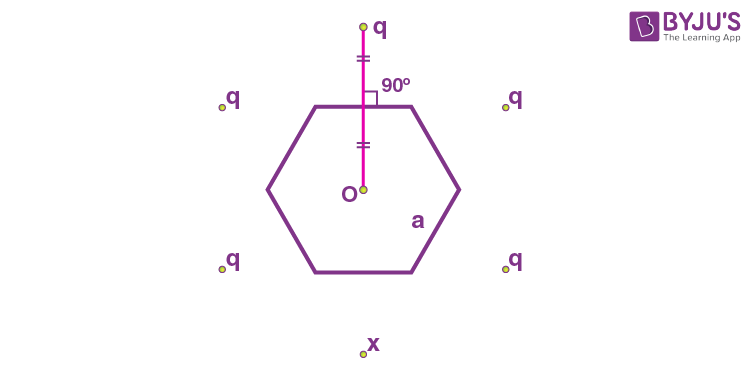Which of the following statement(s) is(are) correct in SI units?

(A) When x = q, the magnitude of the electric field at O is zero.

(B) When x = – q, the magnitude of the electric field at O is

$$\begin{array}{l}\frac{q}{{6\pi { \in _0}{a^2}}}.\end{array}$$

(C) When x = 2q, the potential at O is

$$\begin{array}{l}\frac{{7q}}{{4\sqrt 3 \pi { \in _0}a}}\end{array}$$

(D) When x = – 3q, the potential at O is

$$\begin{array}{l}-\frac{{3q}}{{4\sqrt 3 \pi { \in _0}a}}\end{array}$$

Sol. When x = q, the situation is symmetric

⇒ Electric field at O would be zero.

⇒ (A) is correct.

When x = –q, we can think of x as q + (– 2q) ⇒ Magnitude of electric field at

$$\begin{array}{l}O=\frac{1}{4\pi \in_0}\frac{(2q)}{\left(2 \times \frac{\sqrt 3 a}{2} \right)^2}\end{array}$$
$$\begin{array}{l} = \frac{1}{{4\pi { \in _0}}}\frac{{2q}}{{3{a^2}}} = \frac{q}{{6\pi { \in _0}{a^2}}}\end{array}$$

⇒ (B) is correct

For x = 2q, potential at O is

$$\begin{array}{l}{V_0} = 6 \times \frac{1}{{4\pi { \in _0}}} \times \frac{q}{{\sqrt 3 a}} + \frac{1}{{4\pi { \in _0}}}\frac{q}{{\sqrt 3 a}}\end{array}$$
$$\begin{array}{l} = \frac{{7q}}{{4\sqrt 3 \pi { \in _0}a}}\end{array}$$

⇒ (C) is correct

For x = – 3q,

$$\begin{array}{l}{V_0} = 2 \times \frac{1}{{4\pi { \in _0}}} \times \frac{q}{{\sqrt 3 a}}\end{array}$$
$$\begin{array}{l} = \frac{q}{{2\sqrt 3 \pi { \in _0}a}}\end{array}$$

⇒ (D) is not correct.

14. The binding energy of nucleons in a nucleus can be affected by the pairwise Coulomb repulsion. Assume that all nucleons are uniformly distributed inside the nucleus. Let the binding energy of a proton be Ebp, and the binding energy of a neutron be Ebn in the nucleus.

Which of the following statement(s) is(are) correct?

$$\begin{array}{l}(A)\ E_{b}^p – E_{b}^n\ \text{is proportional to}\ Z(Z-1)\end{array}$$
where Z is the atomic number of the nucleus.

$$\begin{array}{l}(B)\ E_b^p – E_b^n\ \text{is proportional to}\ {A^{ – \frac{1}{3}}}\end{array}$$
where A is the mass number of the nucleus.

$$\begin{array}{l}(C)\ E_b^p – E_b^n\ \text{is positive.}\end{array}$$

(D) Ebp increases if the nucleus undergoes a beta decay emitting a positron.

Sol. Total binding energy (without considering repulsions),

$$\begin{array}{l}{E_b}\; = \;[Z{m_p} + \;(A\;-\;Z){m_n} – \;{m_x}]\;{c^2}\end{array}$$

Where,

$$\begin{array}{l}_Z^AX\ \text{is the nuclei under consideration.}\end{array}$$

Now, considering repulsion :

$$\begin{array}{l}\text{Number of proton pairs }= {^Z}{C_2}\end{array}$$
$$\begin{array}{l}\Rightarrow \text{This repulsion energy} \propto \;\frac{{Z(Z – 1)}}{2} \times \frac{1}{{4\pi \,{ \in _0}}}\;\frac{{{e^2}}}{R}\end{array}$$

Where R is the radius of the nucleus

$$\begin{array}{l}\Rightarrow E_b^p\; – \;E_b^n\; \propto \;Z(Z – 1)\end{array}$$

∴ there will be no repulsion term for neutrons.

Also, since

$$\begin{array}{l}R = {R_0}{A^{1/3}}\end{array}$$
$$\begin{array}{l}\Rightarrow E_b^p – \;E_b^n\; \propto \;{A^{-1/3}}\end{array}$$

Because of repulsion among protons,

$$\begin{array}{l}E_b^p< E_b^n\end{array}$$

Since in β+ decay, number of protons decrease ⇒ repulsion would decrease

$$\begin{array}{l}\Rightarrow E_b^p\ \text{increases}\end{array}$$

SECTION – 3 (Maximum marks : 12)

∙ This section contains FOUR (04) Matching List Sets.

∙ Each set has ONE Multiple Choice Question.

∙ Each set has TWO lists: List-I and List-II.

List-I has Four entries (I), (II), (III) and (IV) and List-II has Five entries (P), (Q), (R), (S) and (T).

∙ FOUR options are given in each Multiple Choice Question based on List-I and List-II and ONLY ONE of these four options satisfies the condition asked in the Multiple Choice Question.

∙ Answer to each question will be evaluated according to the following marking scheme:

Full Marks : +3 ONLY if the option corresponding to the correct combination is chosen;

Zero Marks : 0 If none of the options is chosen (i.e. the question is unanswered);

Negative Marks : −1 In all other cases.

15. A small circular loop of area A and resistance R is fixed on a horizontal xy-plane

$$\begin{array}{l}\text{with the center of the loop always on the axis}\ \hat n\ \text{of a long solenoid. }\end{array}$$
The solenoid has m turns per unit length and carries current I counterclockwise as shown. In the figure.
$$\begin{array}{l}\text{The magnetic field due to the solenoid is in}\ \hat n\ \text{direction}.\end{array}$$
$$\begin{array}{l}\text{List-I gives time dependences of }\ \hat n\end{array}$$
in terms of a constant angular frequency ω. List-II gives the torques experienced by the circular loop at time
$$\begin{array}{l}t = \frac{\pi }{{6\omega }},\ \text{let}\ \theta=\frac{{{A^2}\mu _0^2{m^2}{I^2}\omega }}{{2R}}.\end{array}$$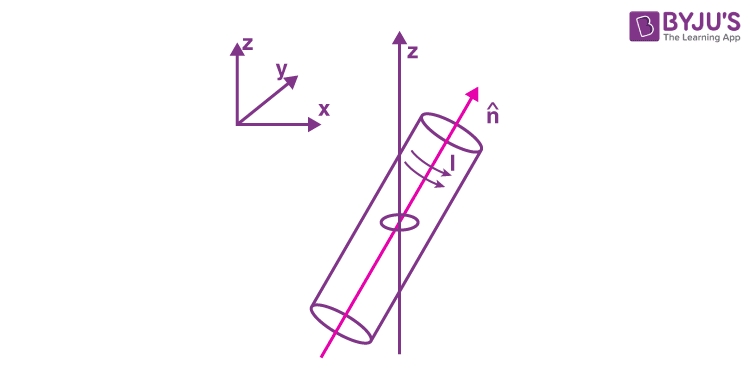List-I List-II
$$\begin{array}{l}(I)\ \frac{1}{{\sqrt 2 }}\left( {\sin \omega t\;\hat j + \cos \omega t\;\hat k} \right)\end{array}$$
(P) 0
$$\begin{array}{l}(II)\ \frac{1}{{\sqrt 2 }}\left( {\sin \omega t\;\hat i + \cos \omega t\;\hat j} \right)\end{array}$$
$$\begin{array}{l} (Q)\ – \frac{\alpha}{4}\hat {i}\end{array}$$
$$\begin{array}{l}(III)\ \frac{1}{{\sqrt 2 }}\left( {\sin \;\omega t\;\hat i + \cos \omega t\;\hat k} \right)\end{array}$$

$$\begin{array}{l}(R)\ \frac{{3\alpha }}{4}\hat i\end{array}$$
$$\begin{array}{l}(IV)\ \frac{1}{{\sqrt 2 }}\left( {\cos \omega t\;\hat j + \sin \;\omega t\;\hat k} \right)\end{array}$$
$$\begin{array}{l}(S)\ \frac{\alpha }{4}\hat j\end{array}$$
$$\begin{array}{l}(T)\ – \frac{{3\alpha }}{4}\hat i\end{array}$$

Which one of the following options is correct?

(A) I → Q, II → P, III → S, IV → T

(B) I → S, II → T, III → Q, IV → P

(C) I → Q, II → P, III → S, IV → R

(D) I → T, II → Q, III → P, IV → R

Answer (C*) (Bonus declared by JEE)

Sol. I.

$$\begin{array}{l}\phi= B A \hat k \cdot \hat n\end{array}$$
$$\begin{array}{l}\phi = \frac{{BA }}{{\sqrt 2 }}\cos \left( {\omega t} \right)\end{array}$$
$$\begin{array}{l}\varepsilon = \frac{{BA \omega }}{{\sqrt 2 }}\sin \left( {\omega t} \right)\end{array}$$
$$\begin{array}{l}i =\frac{{BA\omega }}{{\sqrt 2 R}}\sin \left( {\omega t} \right)\end{array}$$
$$\begin{array}{l}\vec m=iA\;\hat k = \frac{{B{A^2}\omega}}{{\sqrt 2 R}}\sin \left( {\omega t} \right)\,\hat k\end{array}$$
$$\begin{array}{l}\vec{\tau}=\vec m \times \vec B = \frac{{{B^2}{A^2}\omega }}{{\sqrt 2 R}}\sin \left( {\omega t} \right)\left( {\hat k \times \hat n} \right)\end{array}$$
$$\begin{array}{l} =-\frac{{{B^2}{A^2}\omega }}{{2R}}\left[ {\hat i} \right]{\sin ^2}\left( {\omega t} \right)\end{array}$$
$$\begin{array}{l}\tau=-\frac{{{B^2}{A^2}\omega }}{{2R}}\left[ {{{\sin }^2}\left( {\frac{\pi }{6}} \right)} \right] = \frac{{ – \alpha }}{4}\hat i\end{array}$$

(I) → Q.

II. φ = O

(II) → P

III.

$$\begin{array}{l}\phi = \frac{{BA}}{{\sqrt 2 }}\cos \left( {\omega t} \right)\end{array}$$
$$\begin{array}{l}i = \frac{{BA\omega }}{{\sqrt 2 R}}\sin \left( {\omega t} \right)\end{array}$$
$$\begin{array}{l}\vec m = \frac{{B{A^2}\omega }}{{\sqrt 2 R}}\sin \left( {\omega t} \right)\hat k\end{array}$$
$$\begin{array}{l}\vec\tau=\vec m \times \vec B = \frac{B^2A^2\omega}{\sqrt 2\times \sqrt 2 R}\sin \omega t\left({\hat k \times \left( {\sin \omega t\hat i + \cos \omega t\hat k} \right)} \right)\end{array}$$
$$\begin{array}{l}\tau=\frac{{{B^2}{A^2}\omega \sin \left( {\omega t} \right)}}{{2R}}\sin \left( {\omega t} \right)\hat j\end{array}$$
$$\begin{array}{l} = \frac{{{B^2}{A^2}\omega }}{{2R}}{\sin ^2}\left( {\omega t} \right)\hat j\end{array}$$
$$\begin{array}{l} = \frac{\alpha }{4}\hat j\end{array}$$
.

III → S

IV.

$$\begin{array}{l}\phi=\frac{{BA}}{{\sqrt 2 }}\sin \left( {\omega t} \right)\end{array}$$
$$\begin{array}{l}i=- \frac{{BA\omega }}{{\sqrt 2 R}}\cos \left( {\omega t} \right)\end{array}$$
$$\begin{array}{l}\\vec m =-\frac{{B{A^2}\omega }}{{\sqrt 2 R}}\cos \omega t\;\left( {\hat k} \right)\end{array}$$
$$\begin{array}{l}\vec\tau= \vec m\times \vec B =-\frac{{{B^2}{A^2}\omega }}{{2R}}\left( {\hat k \times \hat j} \right){\cos ^2}\left( {\omega t} \right)\end{array}$$
$$\begin{array}{l}\tau=+\frac{{{B^2}{A^2}\omega }}{{2R}}\left( {\hat i} \right) \cdot {\cos ^2}\left( {\frac{\pi }{6}} \right)\end{array}$$
$$\begin{array}{l} =+\frac{3}{4}\alpha \hat i\end{array}$$

(IV) → R.

16. List I describes four systems, each with two particles, A and B, in relative motion, as shown in the figures. List II gives the possible magnitude of their relative velocities (in m s–1) at time t = π/3 s.

List-I List-II
(I) A and B are moving on a horizontal circle of radius 1 m with uniform angular speed ω = 1 rad s–1. The initial angular positions of A and B at time t = 0 are θ = 0 and θ = π/2, respectively.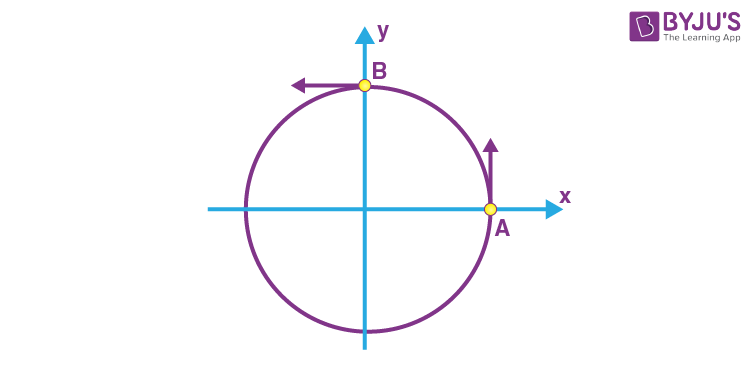(P)
$$\begin{array}{l}\frac{\left(\sqrt3+ 1\right)}{2}\end{array}$$
(II) Projectiles A and B are fired (in the same

vertical plane) at t = 0 and t = 0.1 s respectively,

with the same speed

$$\begin{array}{l}v = \frac{{5\pi }}{{\sqrt 2 }}\,\,{\rm{m}}{{\rm{s}}^{-1}}\end{array}$$
and at 45° from the

horizontal plane. The initial separation between

A and B is large enough so that they do not

collide (g = 10 ms–2)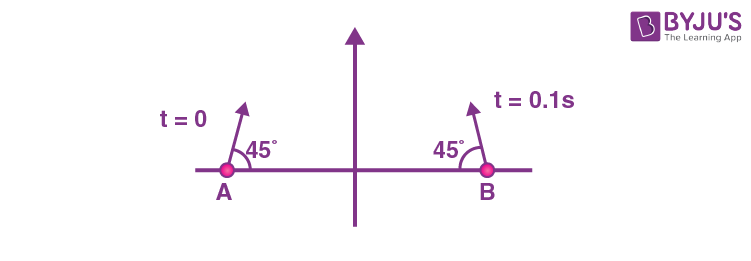(Q)
$$\begin{array}{l}\frac{{\left( {\sqrt 3 -1} \right)}}{{\sqrt 2 }}\end{array}$$
(III) Two harmonic oscillators A and B moving in the x direction according to

$$\begin{array}{l}{x_A} = {x_0}\sin \frac{t}{{{t_0}}}\,{\rm{and}}\,\,{x_B} = {x_0}\sin \left( {\frac{t}{{{t_0}}} + \frac{\pi }{2}} \right)\end{array}$$
respectively, starting from
t = 0. Take x0 = 1 m, t0 = 1 s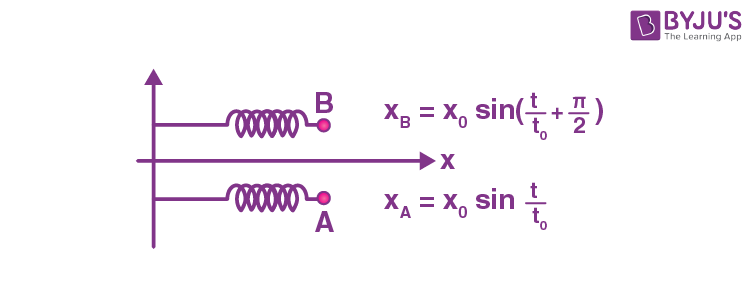(R)
$$\begin{array}{l}\sqrt {10} \end{array}$$
(IV) Particle A is rotating in a horizontal circular path of radius 1 m on the xy plane, with constant angular speed ω = 1 rad s–1. Particle B is moving up at a constant speed 3 ms–1 in the vertical direction as shown in the figure. (Ignore gravity)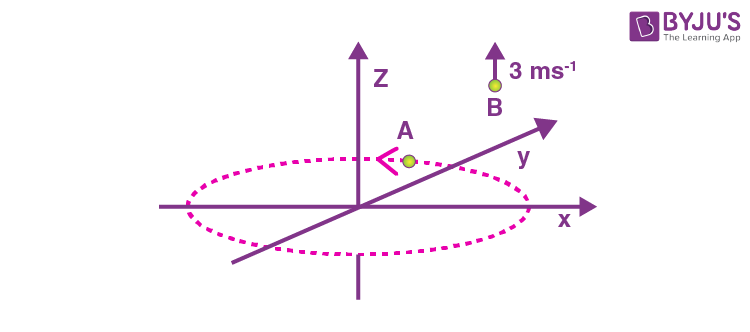(S)
$$\begin{array}{l}\sqrt 2 \end{array}$$
(T)
$$\begin{array}{l}\sqrt {25{\pi ^2} + 1} \end{array}$$

Which one of the following options is correct?

(A) I → R, II → T, III → P, IV → S

(B) I → S, II → P, III → Q, IV → R

(C) I → S, II → T, III → P, IV → R

(D) I → T, II → P, III → R, IV → S

Sol. (I)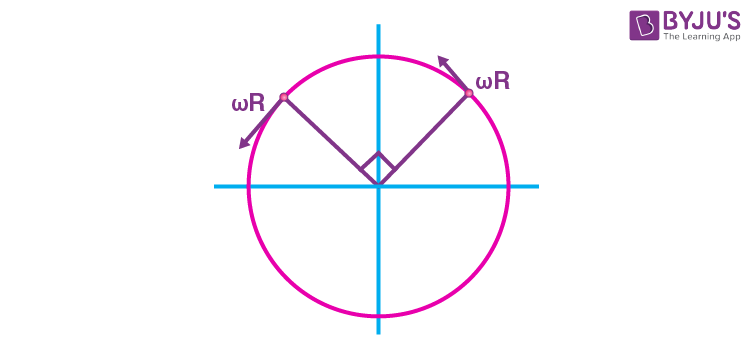$$\begin{array}{l}{v_{{\rm{net}}}} = \sqrt 2 \omega R = \sqrt 2 \end{array}$$

I → S

(II)

$$\begin{array}{l}{v_A}(t = 0.13) = \frac{{5\pi }}{{\sqrt 2 }}\cos 45\;\hat i + \left[ {\frac{{5\pi }}{{\sqrt 2 }} \times \frac{1}{{\sqrt 2 }}-g \times 0.1} \right]\hat j\end{array}$$
$$\begin{array}{l} = \frac{{5\pi }}{2}\hat i + \left( {\frac{{5\pi }}{2}-1} \right)\hat j\end{array}$$
$$\begin{array}{l}{v_B}(t = 0.1\;\sec ) = \frac{{-5\pi }}{2}\hat i + \left( {\frac{{5\pi }}{2}} \right)\hat j\end{array}$$

After t = 0.1, relative velocities should not change.

$$\begin{array}{l}{v_{{\rm{rel}}}}(t = 0.1\;\sec ) = \left| {5\pi \hat i – \hat j} \right|\end{array}$$
$$\begin{array}{l} = \sqrt {25{\pi ^2} + 1} \end{array}$$

II → T

(III) x = xAxB

$$\begin{array}{l} = {x_0}\sin t-{x_0}\sin \left( {t + \frac{\pi }{2}} \right)\end{array}$$
$$\begin{array}{l} = \sqrt 2 {x_0}\sin \left( {t-\frac{\pi }{4}} \right)\end{array}$$
$$\begin{array}{l}{v_{{\rm{rel}}}} = \frac{{dx}}{{dt}} = \sqrt 2 {x_0}\cos \left( {t-\frac{\pi }{4}} \right)\end{array}$$
$$\begin{array}{l} = \sqrt 2 \cos \left( {\frac{\pi }{3}-\frac{\pi }{4}} \right)\end{array}$$
$$\begin{array}{l} =\sqrt{2}\times \frac{\sqrt{3}+ 1}{2\sqrt{2}}=\frac{\sqrt{3}+1}{2}\end{array}$$

III → P

(IV)

$$\begin{array}{l}v_{rel} =\sqrt{3^2+1^2}=\sqrt{10} \end{array}$$

IV → R

17. List I describes thermodynamic processes in four different systems. List II gives the magnitudes (either exactly or as a close approximation) of possible changes in the internal energy of the system due to the process.

List-I List-II
(I) 10–3 kg of water 100°C is converted to steam at the same temperature, at a pressure of 105 Pa. The volume of the system changes from 10–6 m3 to 10–3 m3 in the process. Latent heat of water = 2250 kJ/kg. (P) 2 kJ
(II) 0.2 moles of a rigid diatomic ideal gas with volume V at temperature 500 K undergoes an isobaric expansion to volume 3 V. Assume R = 8.0 J mol–1 K–1. (Q) 7 kJ
(III) One mole of a monoatomic ideal gas is compressed adiabatically from volume
$$\begin{array}{l}V = \frac{1}{3}\ m^3\end{array}$$
and pressure 2 kPa to volume V/8.
(R) 4 kJ
(IV) Three moles of a diatomic ideal gas whose molecules can vibrate, is given 9 kJ of heat and undergoes isobaric expansion. (S) 5 kJ
(T) 3 kJ

Which one of the following options is correct?

(A) I → T, II → R, III → S, IV → Q

(B) I → S, II → P, III → T, IV → P

(C) I → P, II → R, III → T, IV → Q

(D) I → Q, II → R, III → S, IV → T

Sol. (I) U = MLPΔV

= 10–3 × 2250 – 102kP × (10–3 – 10–6) m3

= 2.25 kJ – 0.1 kJ

= 2.15 kJ

I → P

(II)

$$\begin{array}{l}{C_V} = \frac{{5R}}{2}\,\,({\rm{rigid}}\,\,{\rm{diatomic}})\end{array}$$

For isobasic expansion

VT

$$\begin{array}{l}\frac{V_1}{V_2}=\frac{T_1}{T_2}\Rightarrow \frac{V}{3V}=\frac{500}{T_2}\Rightarrow T_2=1500\ K\end{array}$$

$$\begin{array}{l}\Delta U = n{C_V}\Delta T = 0.2 \times \frac{{5 \times 8}}{2} \times (1500-500)\,\,{\rm{J}}\\= 4 ~\text{kJ} \end{array}$$

II → R

$$\begin{array}{l}\left( {\gamma = \frac{5}{3}} \right)\end{array}$$
$$\begin{array}{l}{P_1}\cdot V_1^\gamma={P_2}V_2^\gamma\Rightarrow (2\,\,{\rm{kPa}}) \times V_0^{5/3} = {P_2} \times {\left( {\frac{{{V_0}}}{8}} \right)^{5/3}}\end{array}$$

P2 = 64 kPa

ΔU = nCVΔT

$$\begin{array}{l} = \frac{{3nR\Delta T}}{2} = \frac{3}{2}({P_2}{V_2}-{P_1}{V_1}) = \frac{3}{2} \times \left( {64 \times \frac{1}{{3 \times 8}}-2 \times \frac{1}{3}} \right)\end{array}$$
$$\begin{array}{l} = \frac{3}{2} \times \left( {\frac{8}{3}-\frac{2}{3}} \right) = 3\,\,{\rm{kJ}}\end{array}$$

III → T

(IV) For isobaric expansion,

$$\begin{array}{l}\Delta U = nC_V\Delta T = \frac{7}{2} nR \Delta T\end{array}$$
$$\begin{array}{l}\Delta Q = nC_P\Delta T = \frac{9}{2} nR\Delta T\end{array}$$
$$\begin{array}{l}\frac{{\Delta U}}{{\Delta Q}} = \frac{7}{9}\end{array}$$
$$\begin{array}{l}\Delta U = \frac{7}{9}\Delta Q = 7{\rm{ kJ}}\end{array}$$

IV → Q

18. List I contains four combinations of two lenses (1 and 2) whose focal lengths (in cm) are indicated in the figures. In all cases, the object is placed 20 cm from the first lens on the left, and the distance between the two lenses is 5 cm. List II contains the positions of the final images.

List I List II
(I)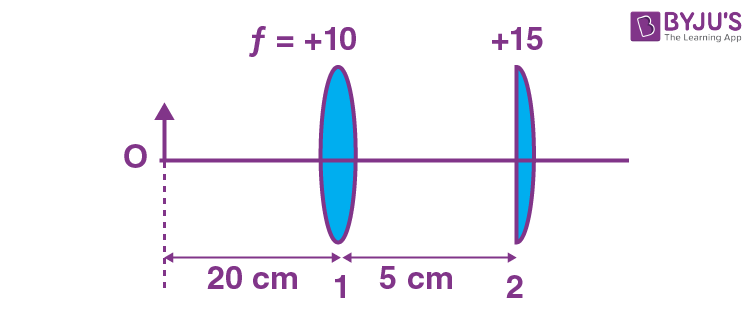(P) Final image is formed at 7.5 cm on the right side of lens 2.
(II)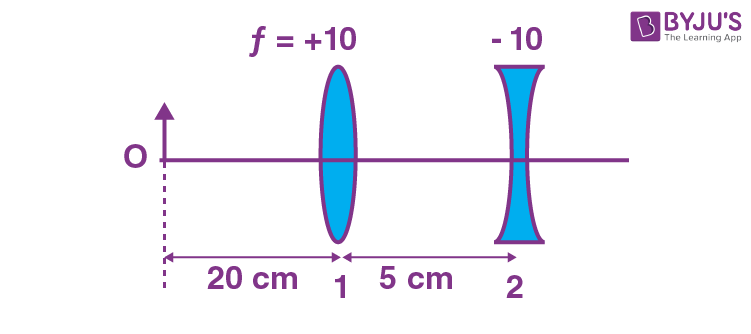(Q) Final image is formed at 60.0 cm on the right side of lens 2.
(III)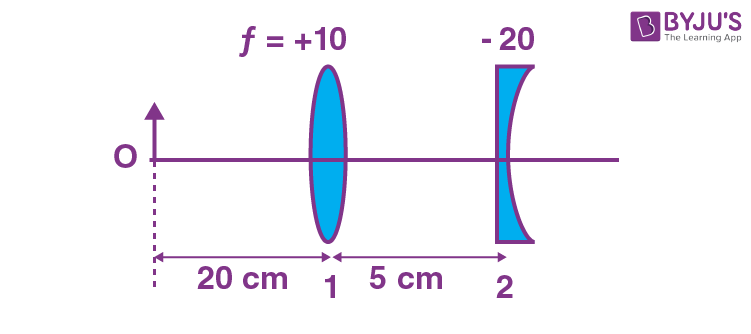(R) Final image is formed at 30.0 cm on the left side of lens 2.
(IV)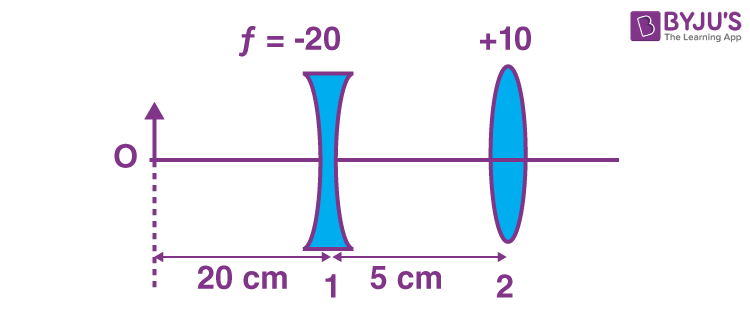(S) Final image is formed at 6.0 cm on the right side of lens 2.
(T) Final image is formed at 30.0 cm on the right side of lens 2.

Which one of the following options is correct?

(A) (I) → P; (II) → R; (III) → Q; (IV) → T

(B) (I) → Q; (II) → P; (III) → T; (IV) → S

(C) (I) → P; (II) → T; (III) → R; (IV) → Q

(D) (I) → T; (II) → S; (III) → Q; (IV) → R

Sol.

(I) u = –20 cm

f = +10 cm

$$\begin{array}{l}\frac{1}{v} + \frac{1}{{20}} = \frac{1}{10}\end{array}$$
$$\begin{array}{l}\frac{1}{v} = \frac{1}{10}-\frac{1}{20}\end{array}$$
$$\begin{array}{l}\frac{1}{v} = \frac{1}{20}\end{array}$$

v = 20 cm

u = +15 cm

f = +15 cm

$$\begin{array}{l}\frac{1}{v}-\frac{1}{u} = \frac{1}{f}\end{array}$$
$$\begin{array}{l}\Rightarrow \frac{1}{v}-\frac{1}{15} = \frac{1}{15}\end{array}$$
$$\begin{array}{l}\frac{1}{v} = \frac{2}{15}\end{array}$$

v = 7.5 cm (from lens 2)

I → P

(II) u = –20 cm, f = +10 cm

$$\begin{array}{l}\frac{1}{v} + \frac{1}{20} = \frac{1}{10}\end{array}$$
$$\begin{array}{l}\frac{1}{v} = \frac{1}{10}-\frac{1}{20} \Rightarrow v = 20\ cm\end{array}$$

u = +15 cm

f = –10 cm

$$\begin{array}{l}\frac{1}{v}-\frac{1}{u} = \frac{1}{f} \Rightarrow \frac{1}{v}-\frac{1}{15} = \frac{-1}{10}\end{array}$$
$$\begin{array}{l}\frac{1}{v} = \frac{-1}{10} + \frac{1}{15} = \frac{-3 + 2}{30}\end{array}$$
$$\begin{array}{l}\frac{1}{v} = -\frac{1}{30}\end{array}$$

v = –30 cm

II → R

(III) u = –20

f = +10 cm

$$\begin{array}{l}\frac{1}{v} + \frac{1}{20} = \frac{1}{10}\end{array}$$
$$\begin{array}{l}\frac{1}{v} = \frac{1}{10}-\frac{1}{20} = \frac{1}{20}\end{array}$$

v = 20 cm

$$\begin{array}{l}\Rightarrow u = 15\ cm\end{array}$$

f = – 20 cm

$$\begin{array}{l}\frac{1}{v}-\frac{1}{15} = \frac{-1}{20}\end{array}$$
$$\begin{array}{l}\frac{1}{v} = -\frac{1}{20} + \frac{1}{15} = \frac{-3 + 4}{60} = \frac{1}{60}\end{array}$$

v = 60 cm

III → Q

(IV) u = –20 cm

f = –20

$$\begin{array}{l} ]\Rightarrow \frac{1}{v}-\frac{1}{u} = \frac{1}{f}\end{array}$$
$$\begin{array}{l} ]\Rightarrow \frac{1}{v} + \frac{1}{20} = \frac{-1}{20}\end{array}$$

v = –10 cm

u = –15 cm

f = 10 cm

$$\begin{array}{l}\frac{1}{v}-\frac{1}{u} = \frac{1}{f}\end{array}$$
$$\begin{array}{l} \Rightarrow \frac{1}{v} + \frac{1}{15} = \frac{1}{10}\end{array}$$
$$\begin{array}{l} \Rightarrow \frac{1}{v} = \frac{1}{10}-\frac{1}{15}\end{array}$$
$$\begin{array}{l} = \frac{3-2}{30} = \frac{1}{30}\end{array}$$

v = 30 cm

IV → T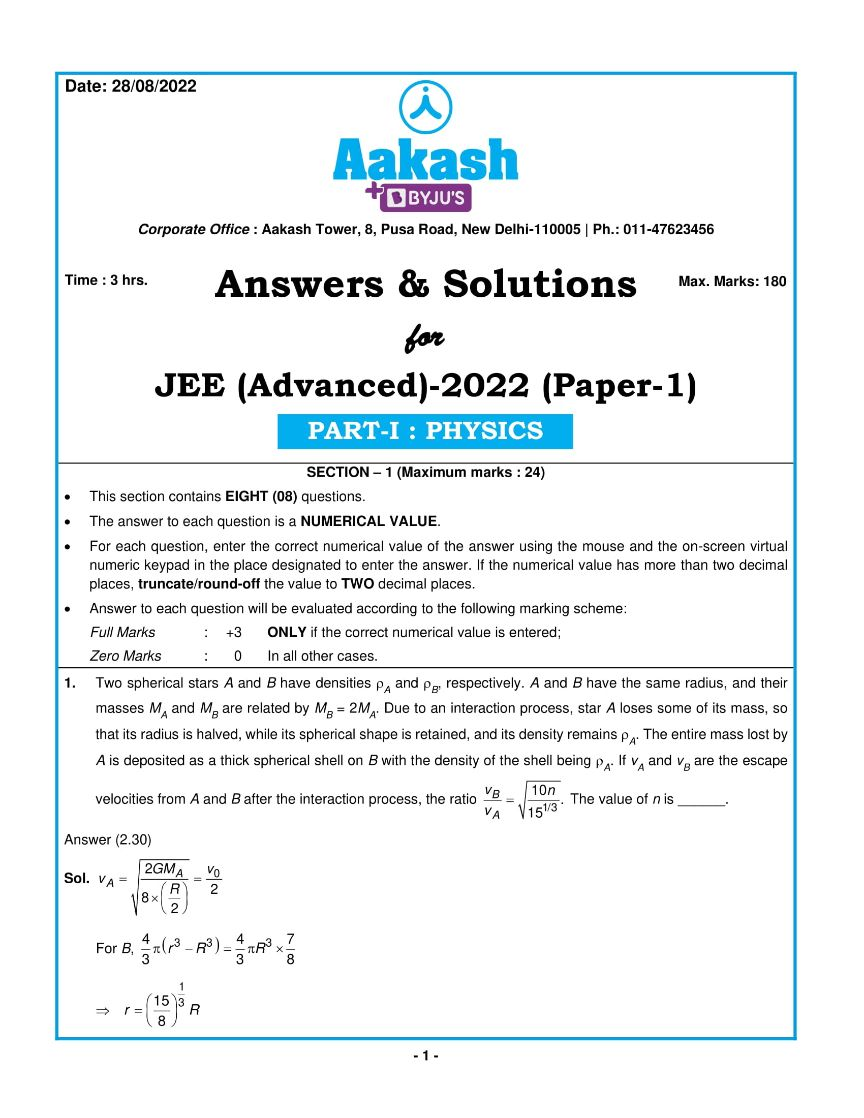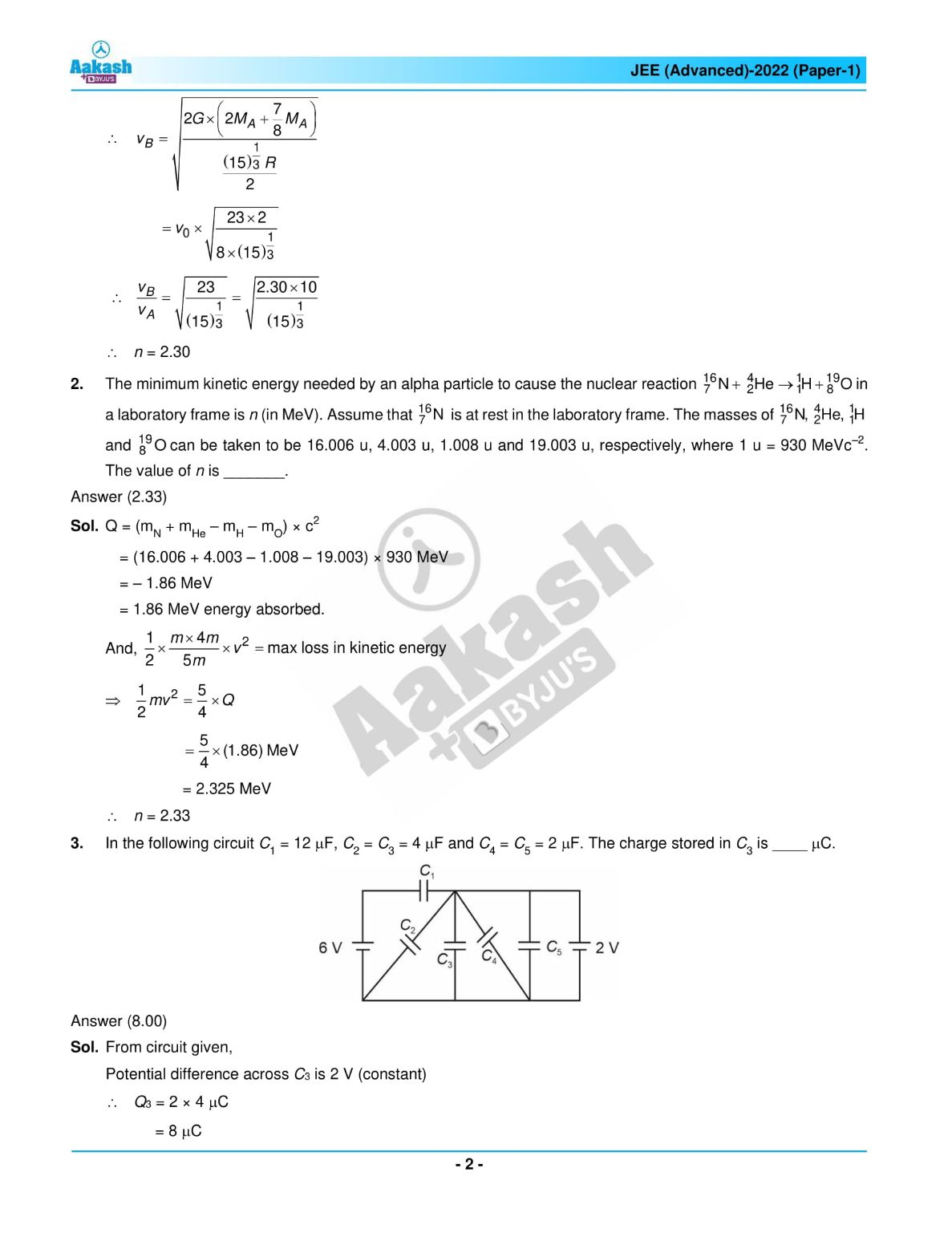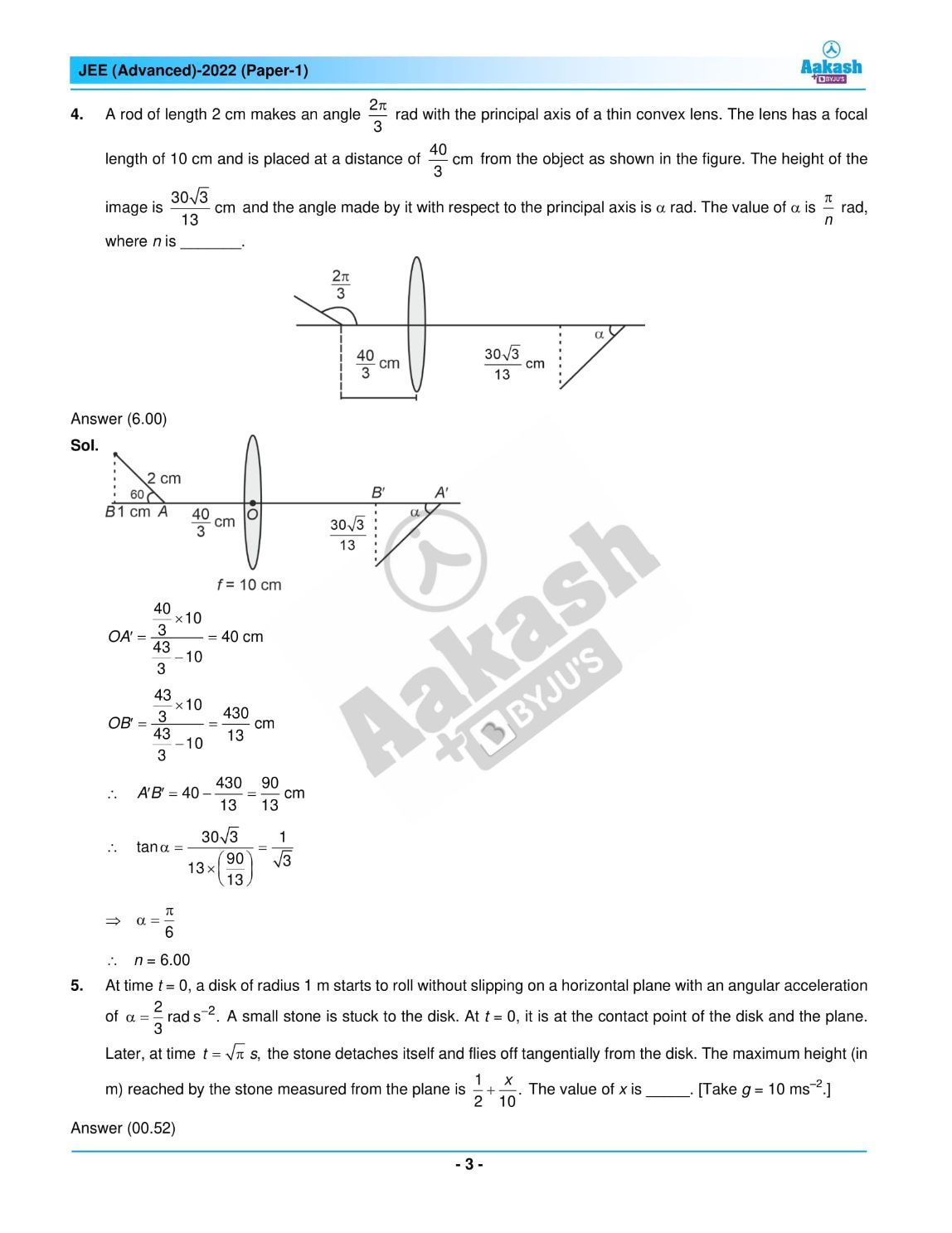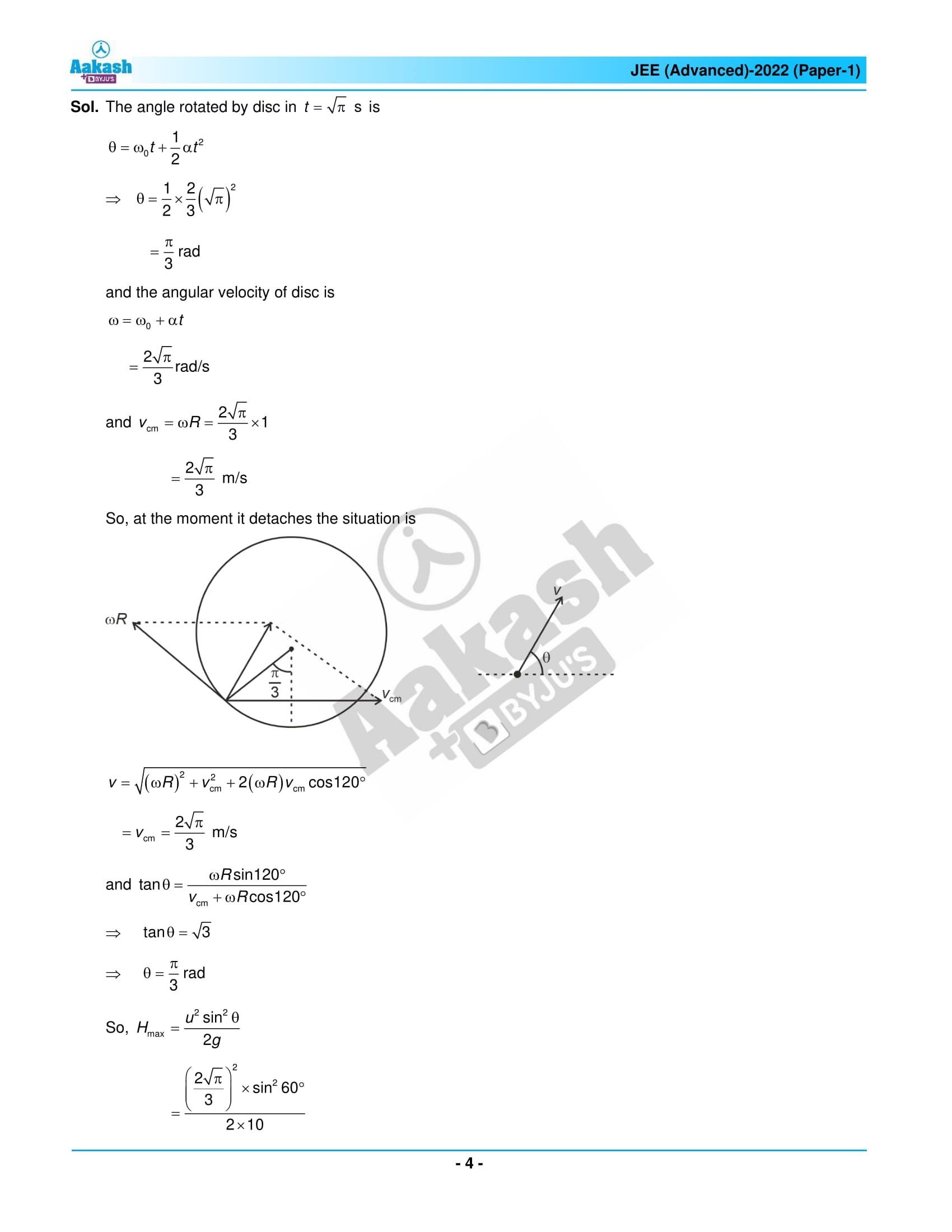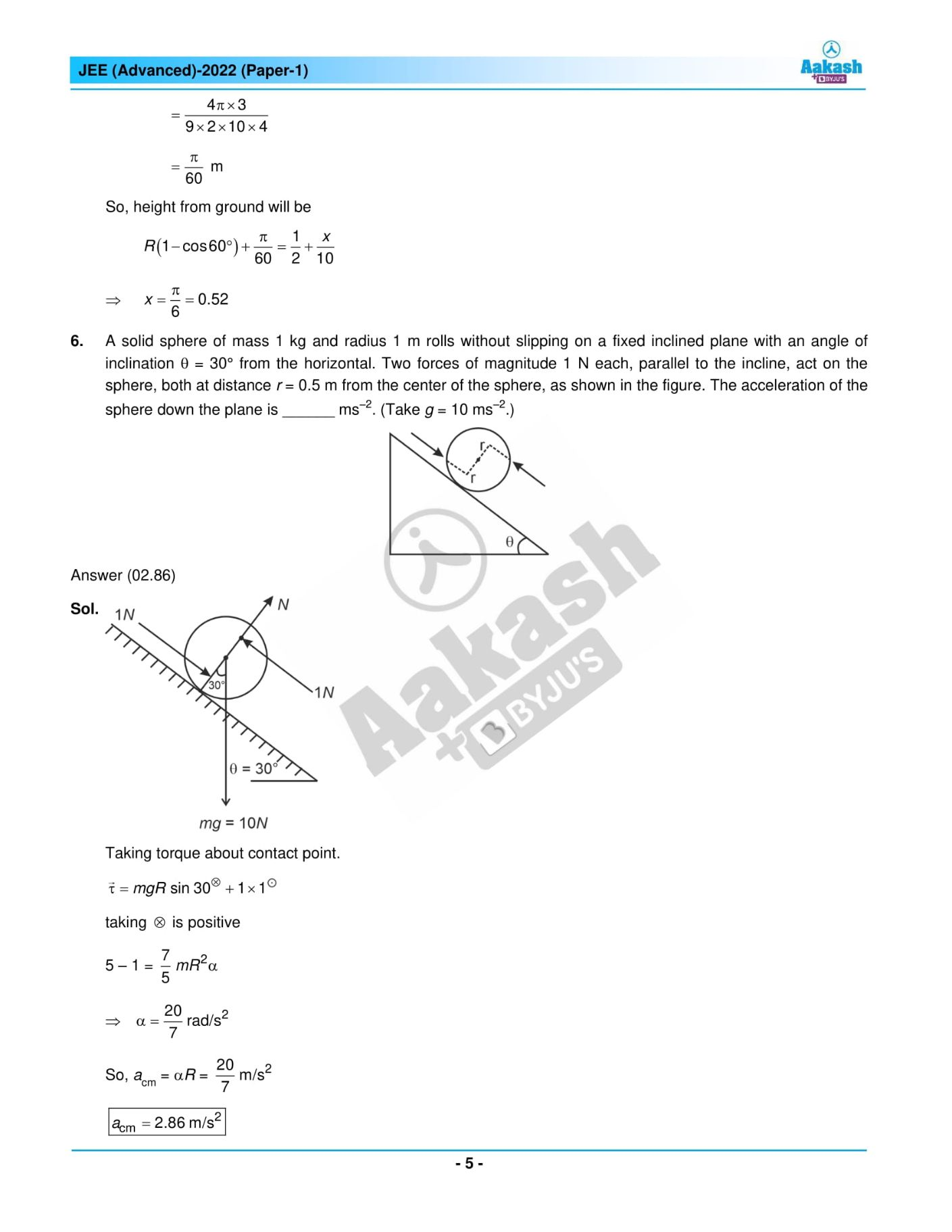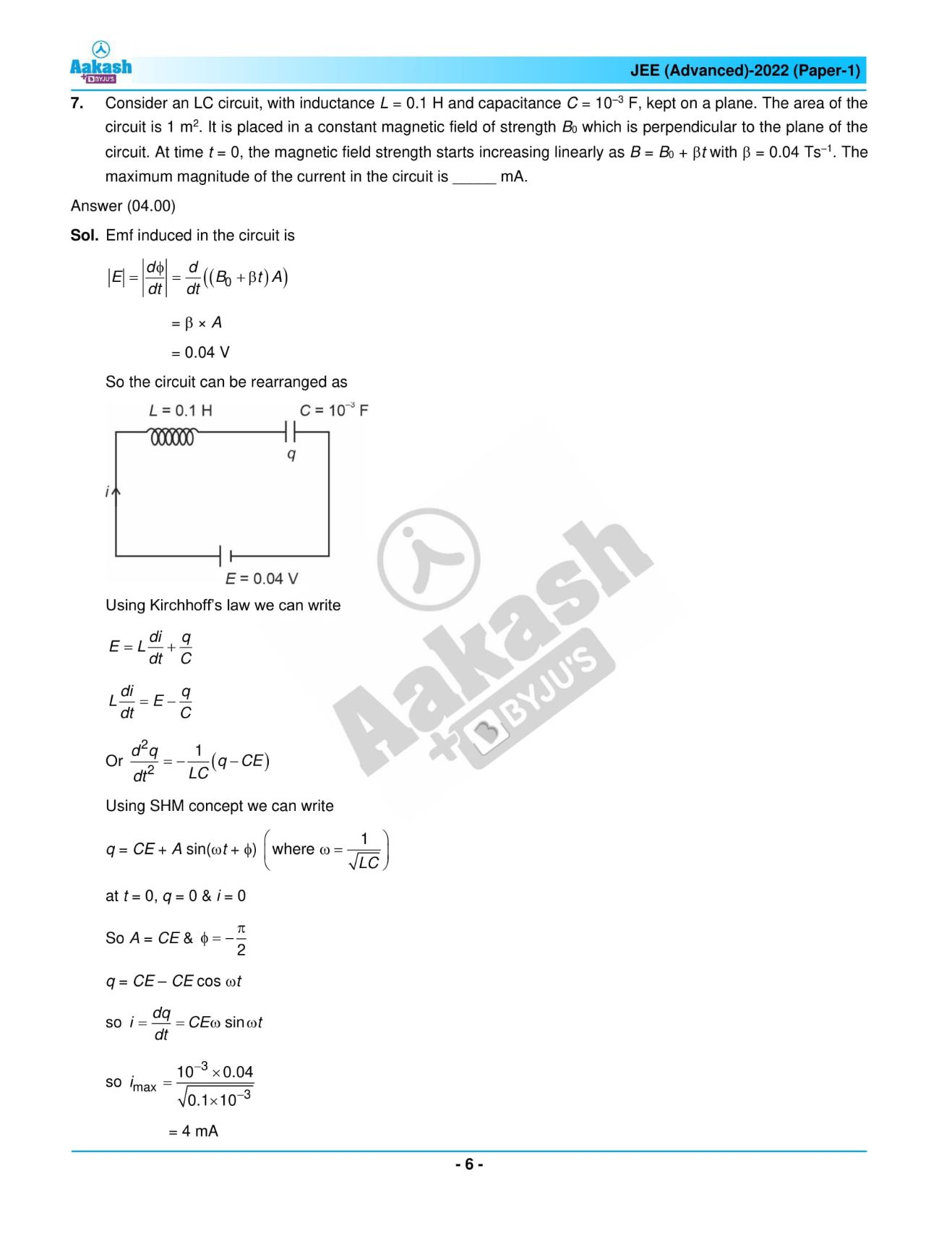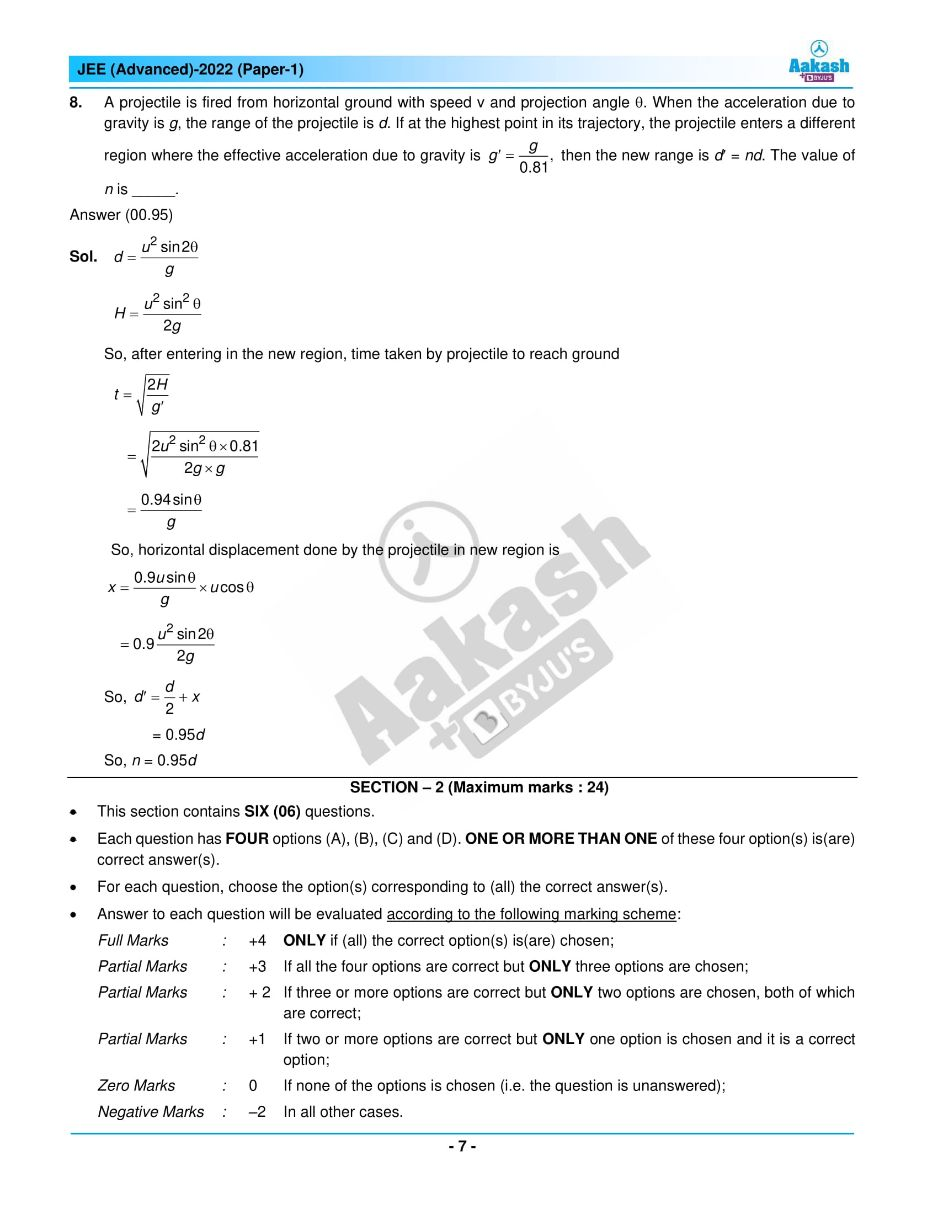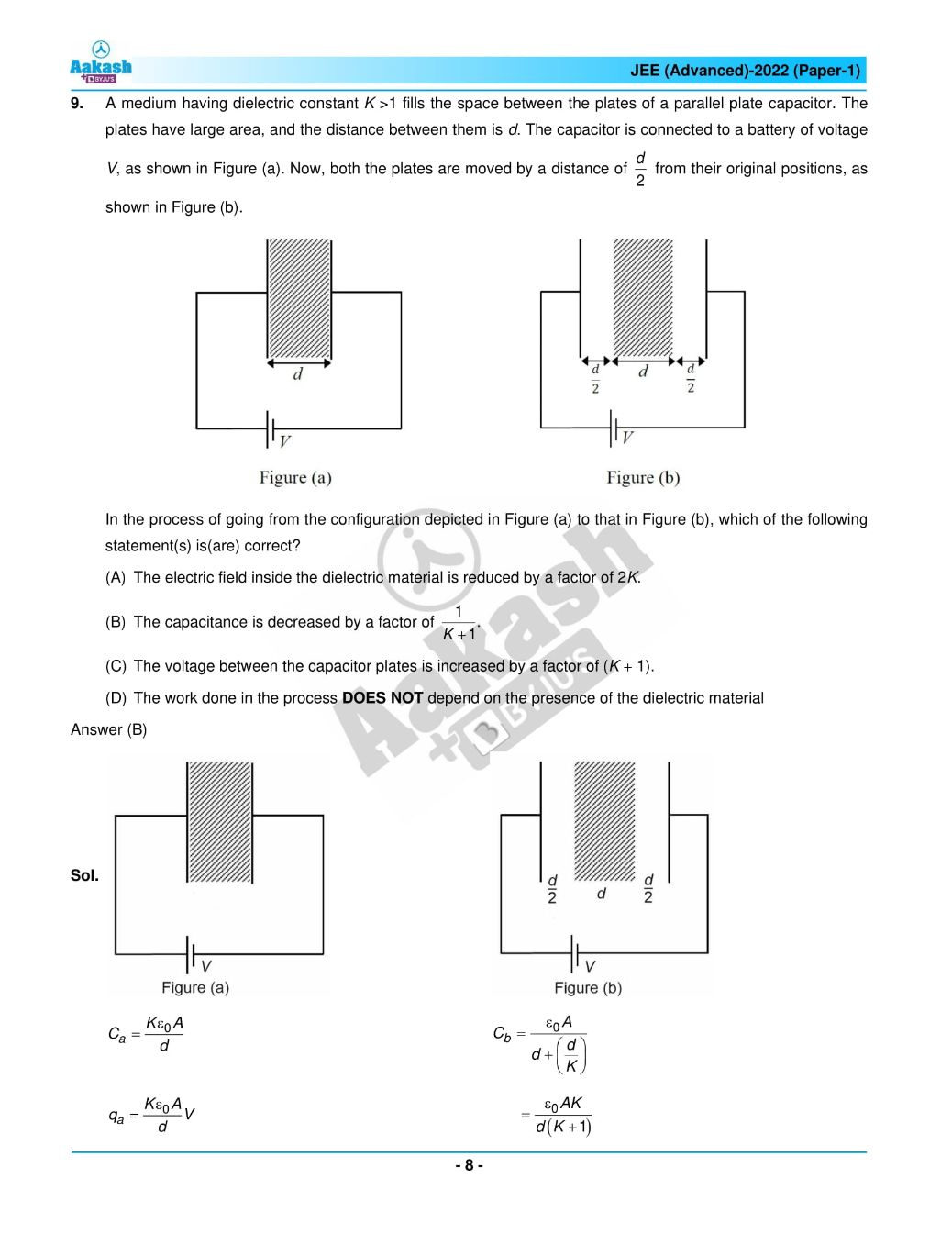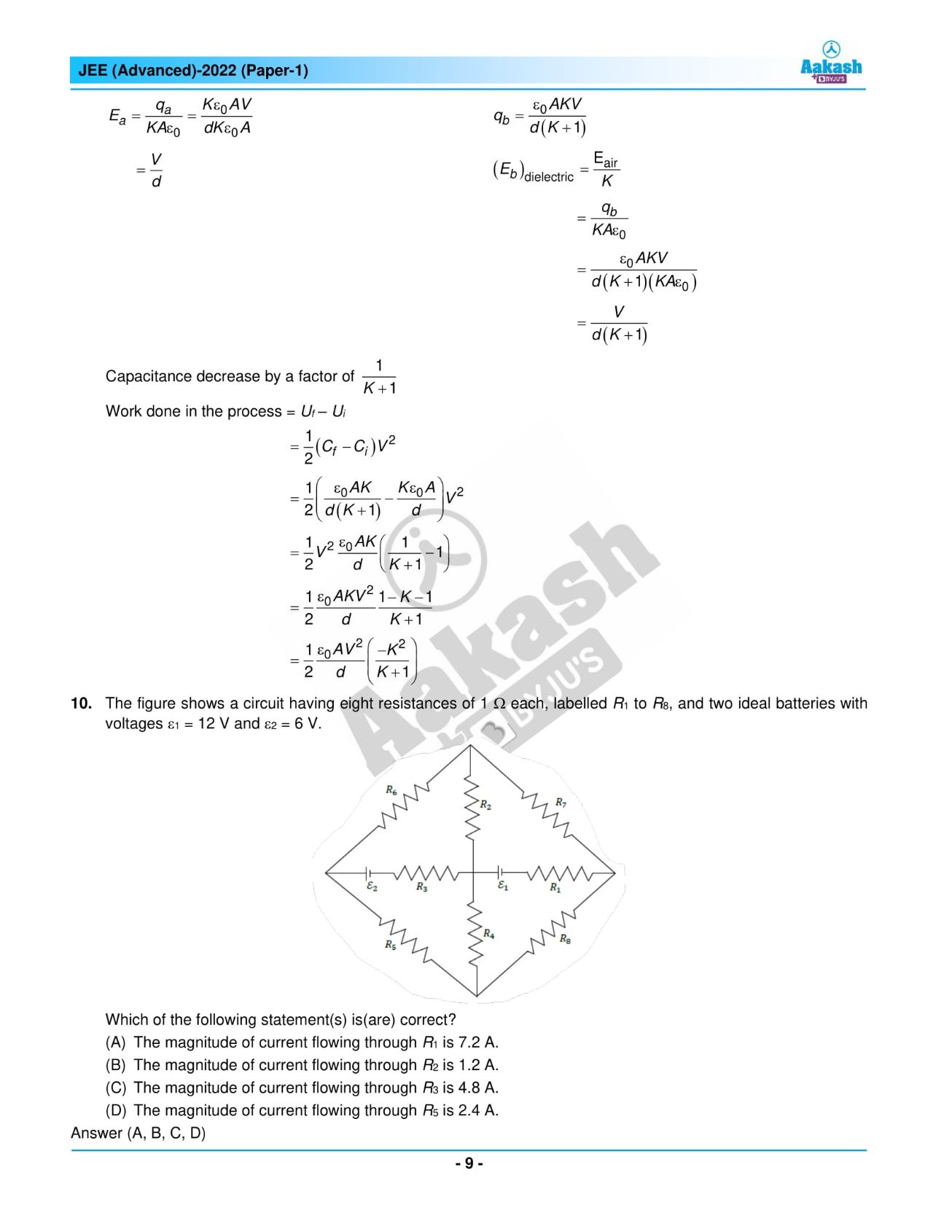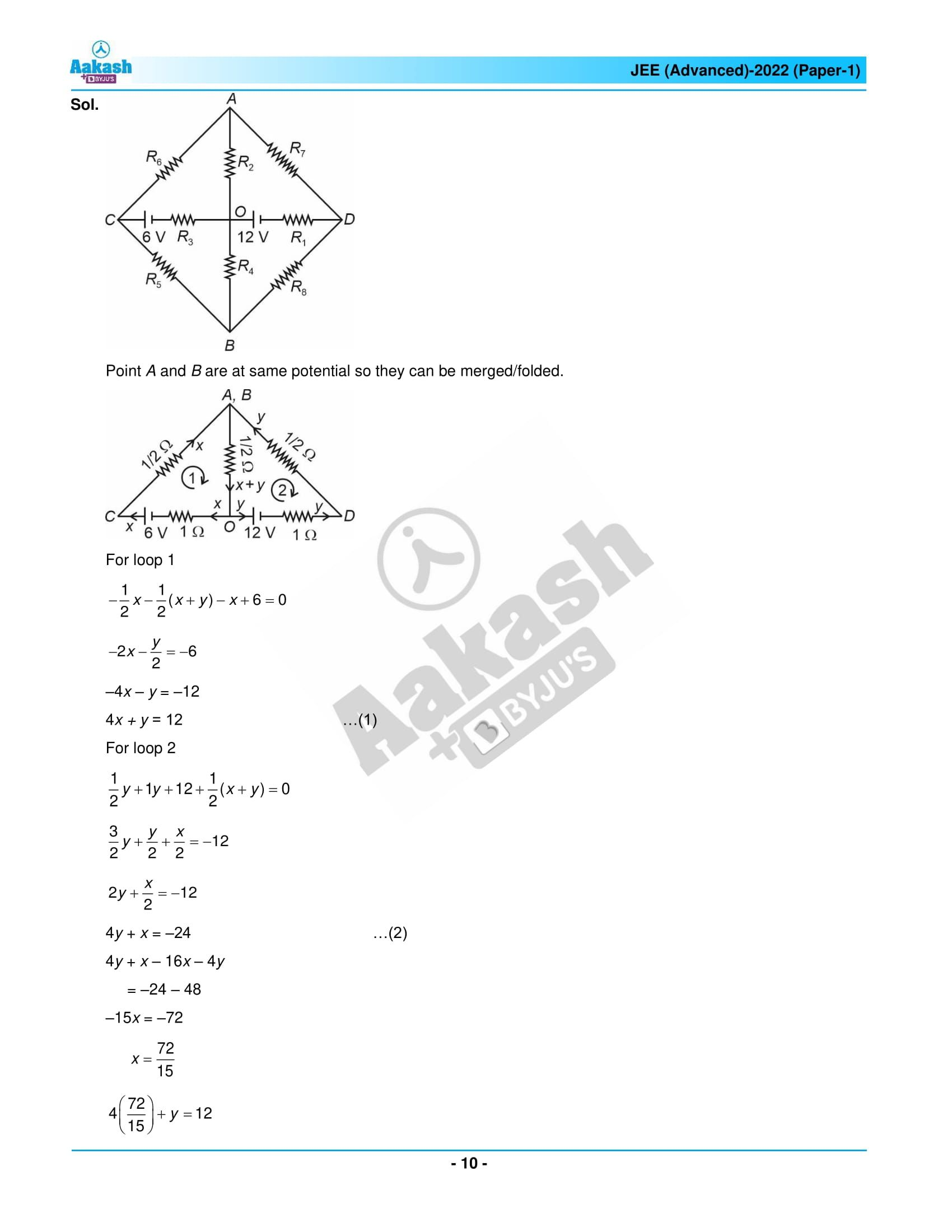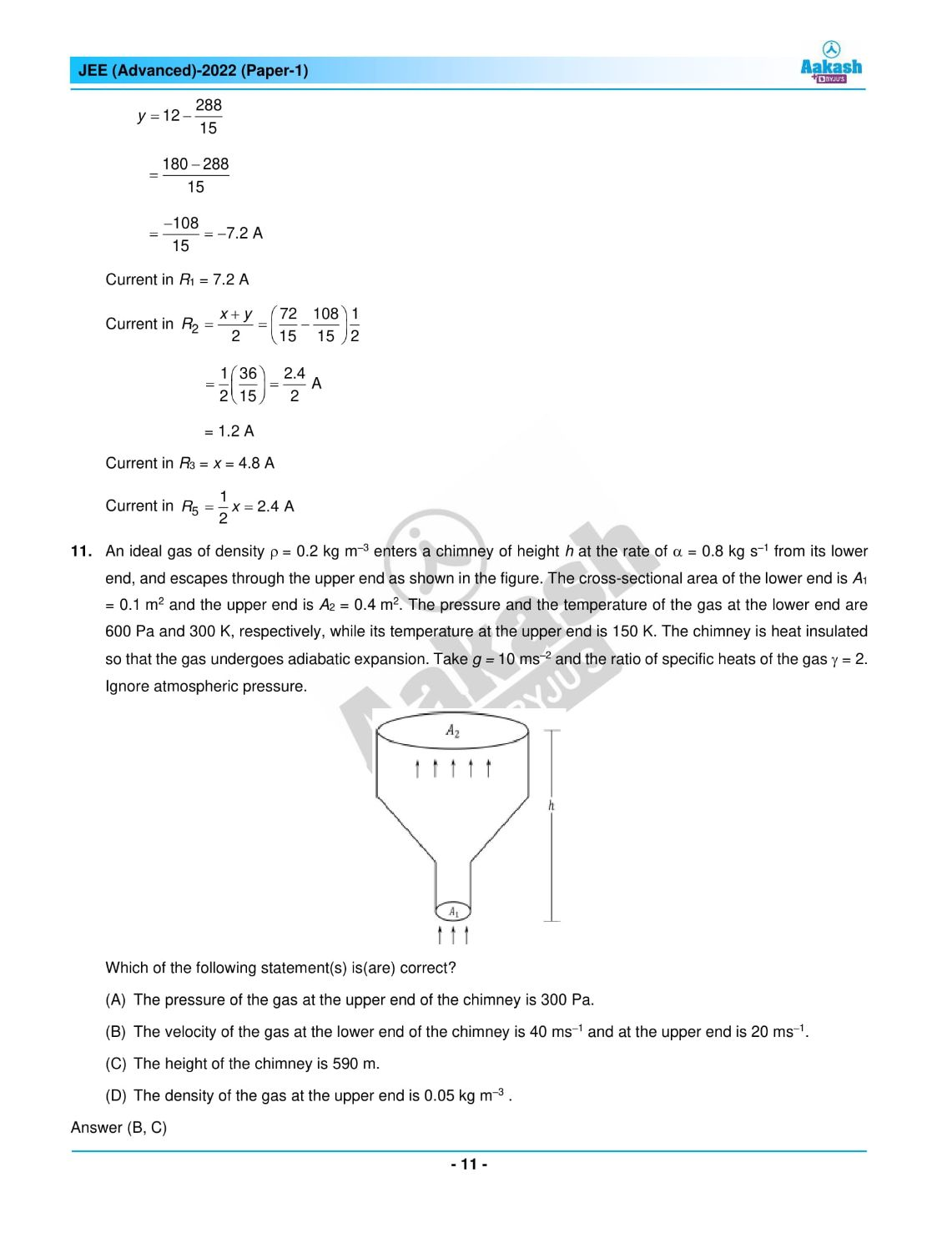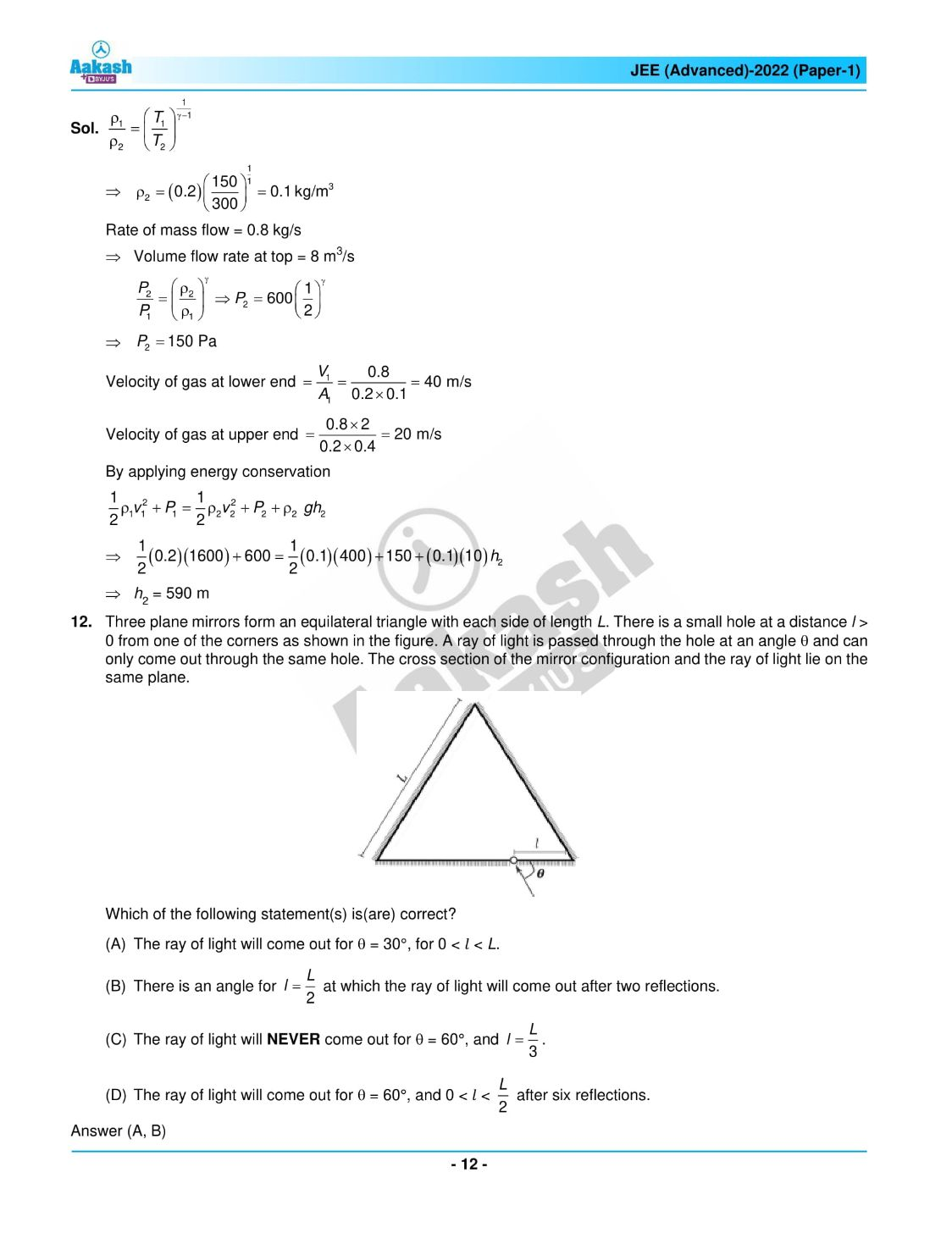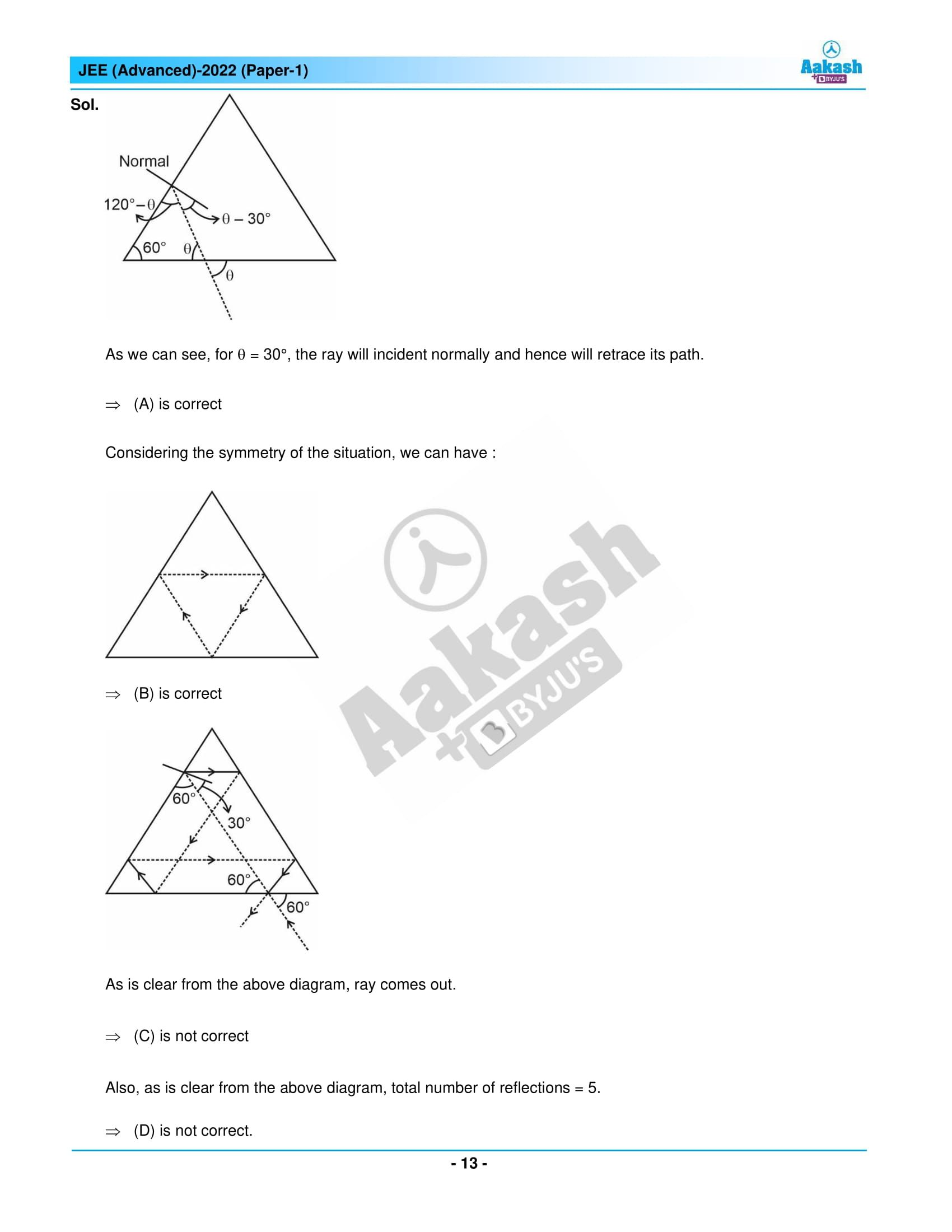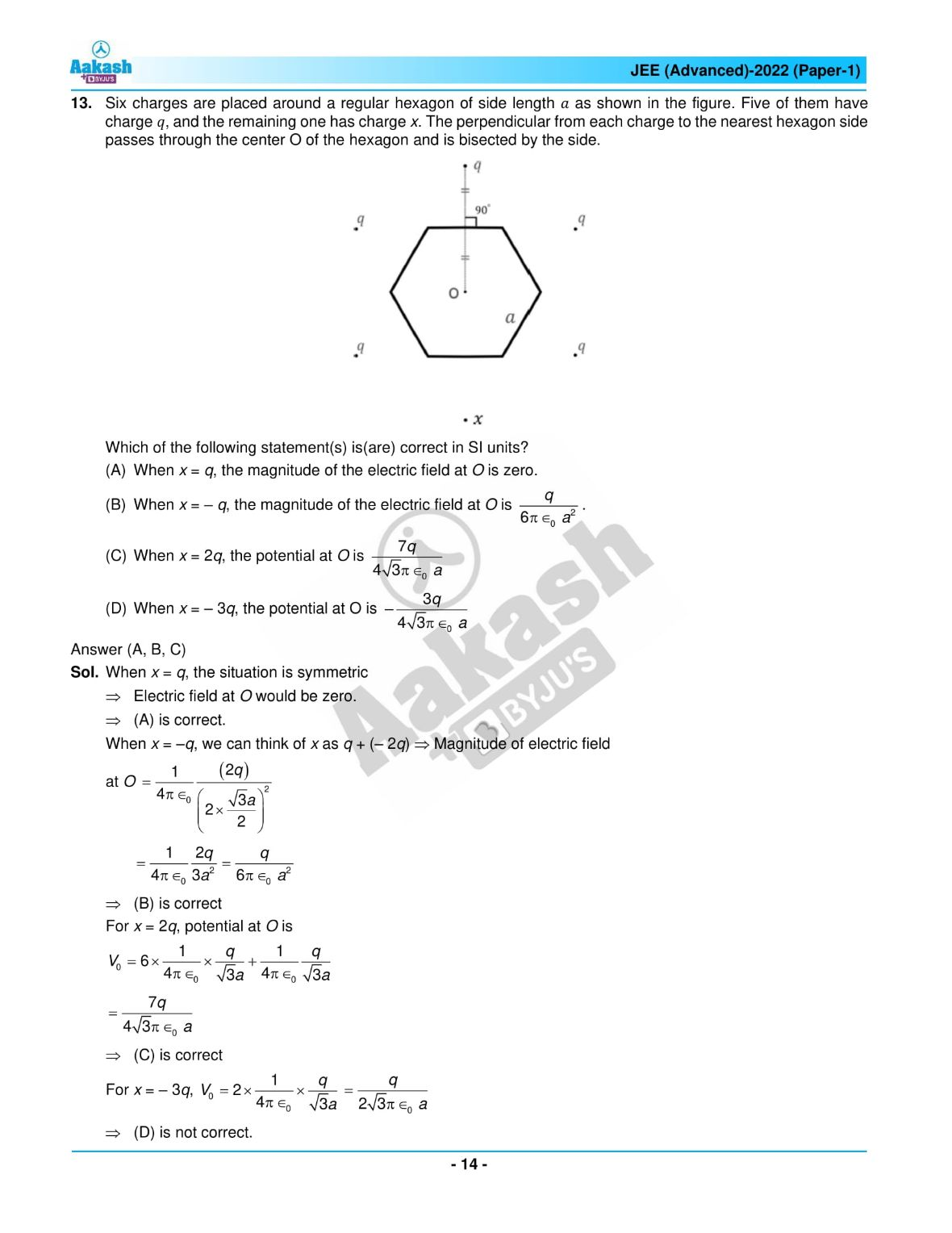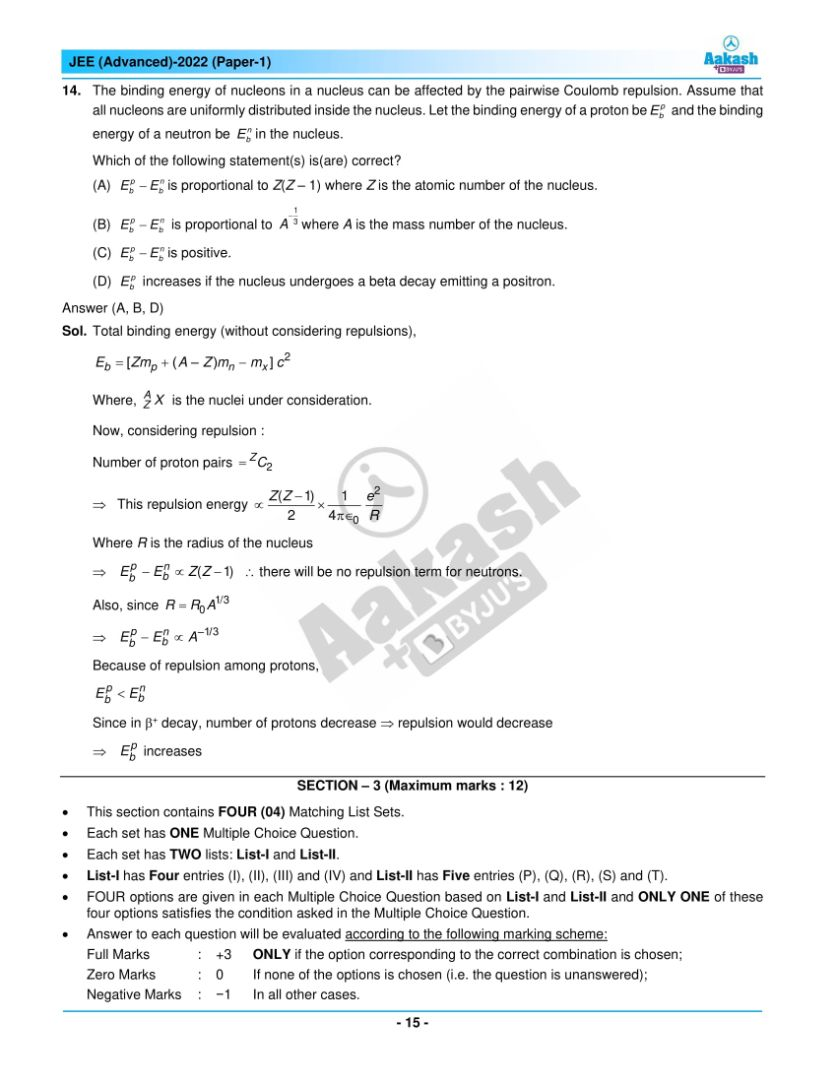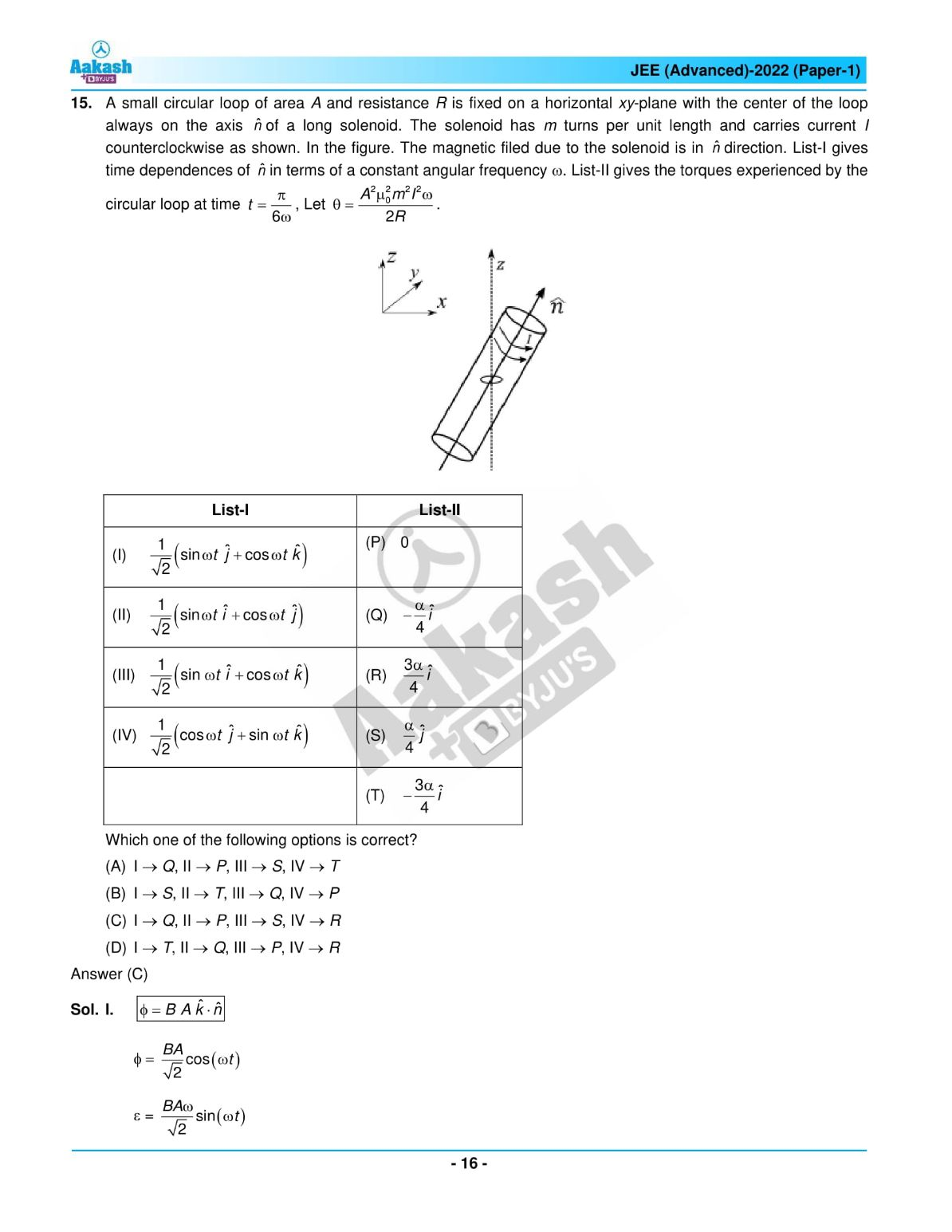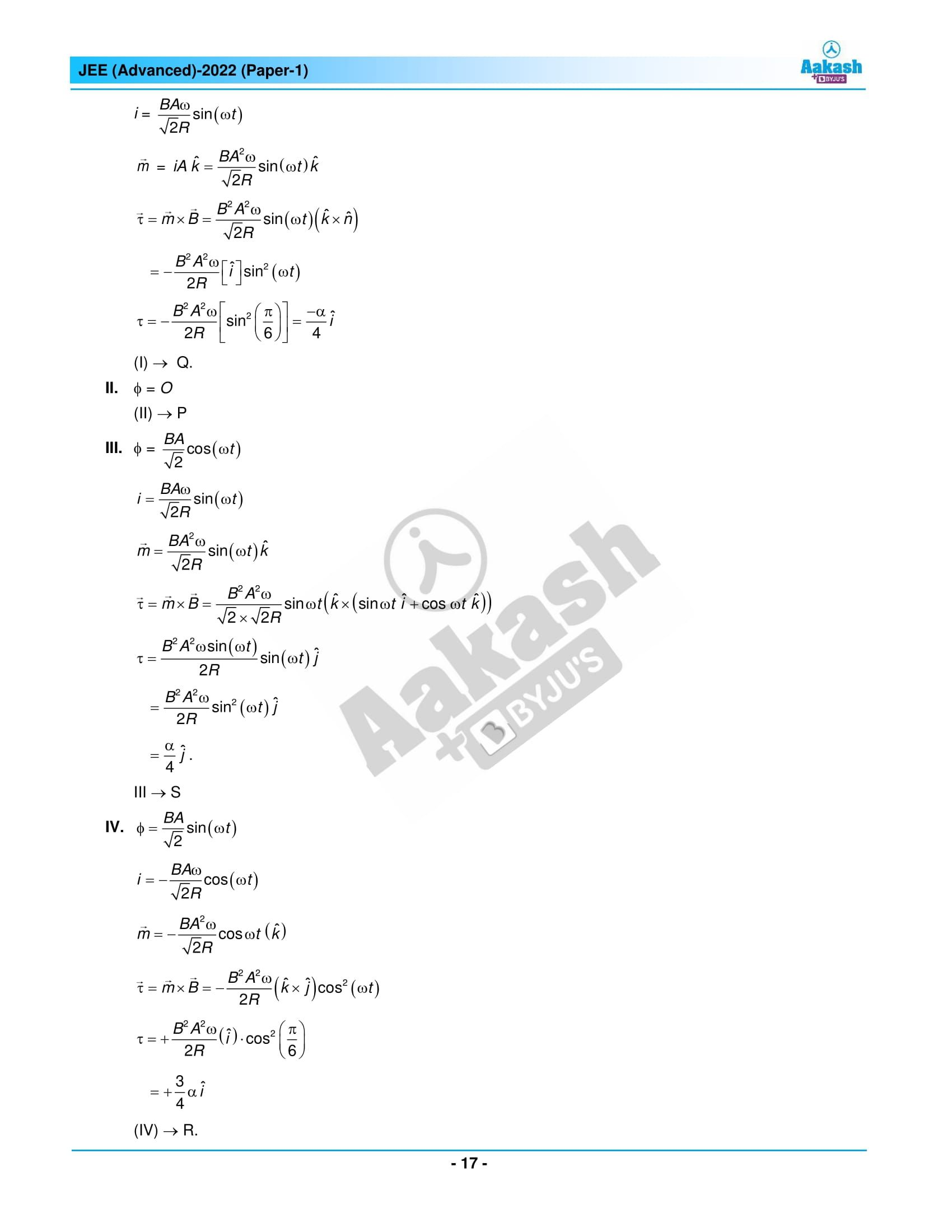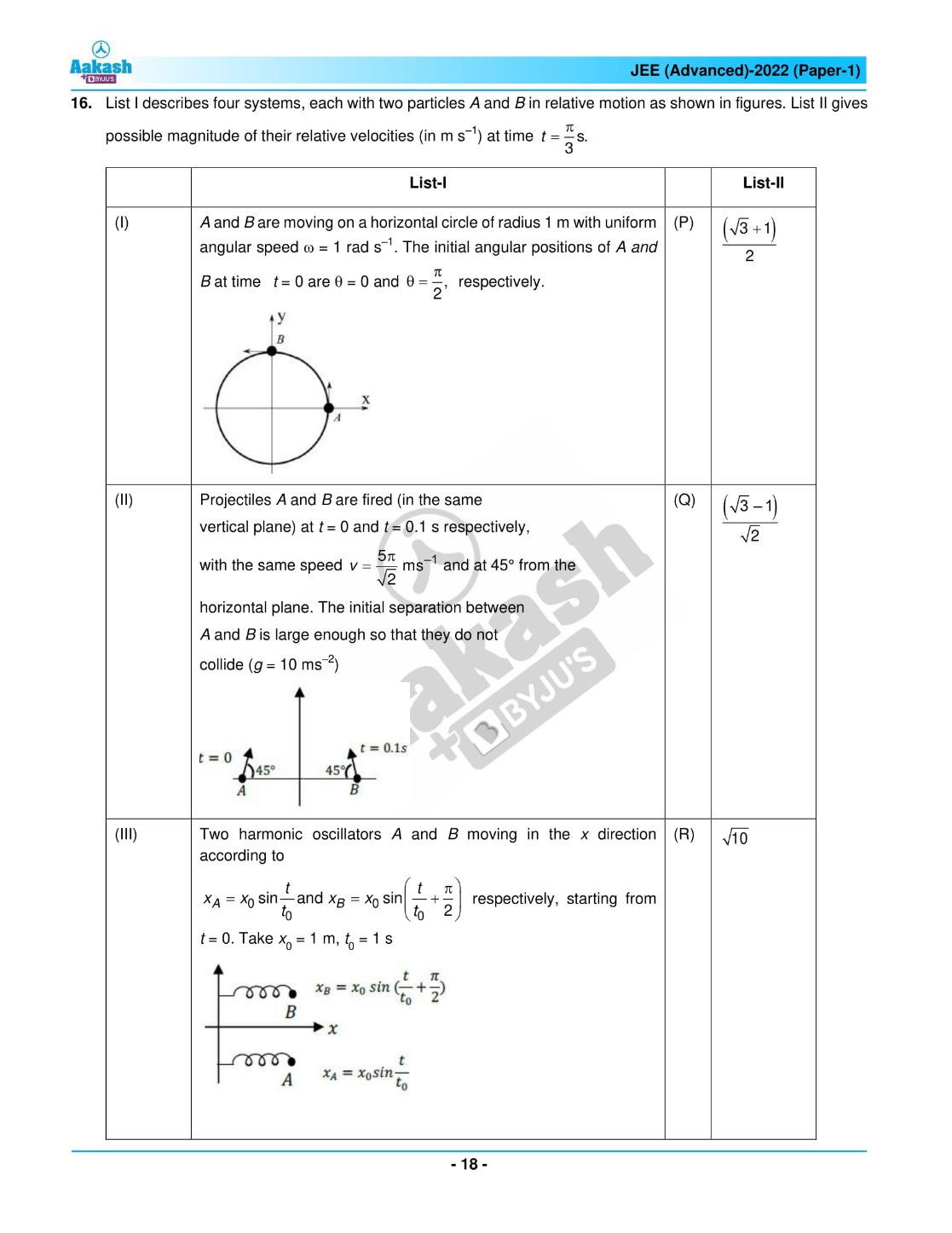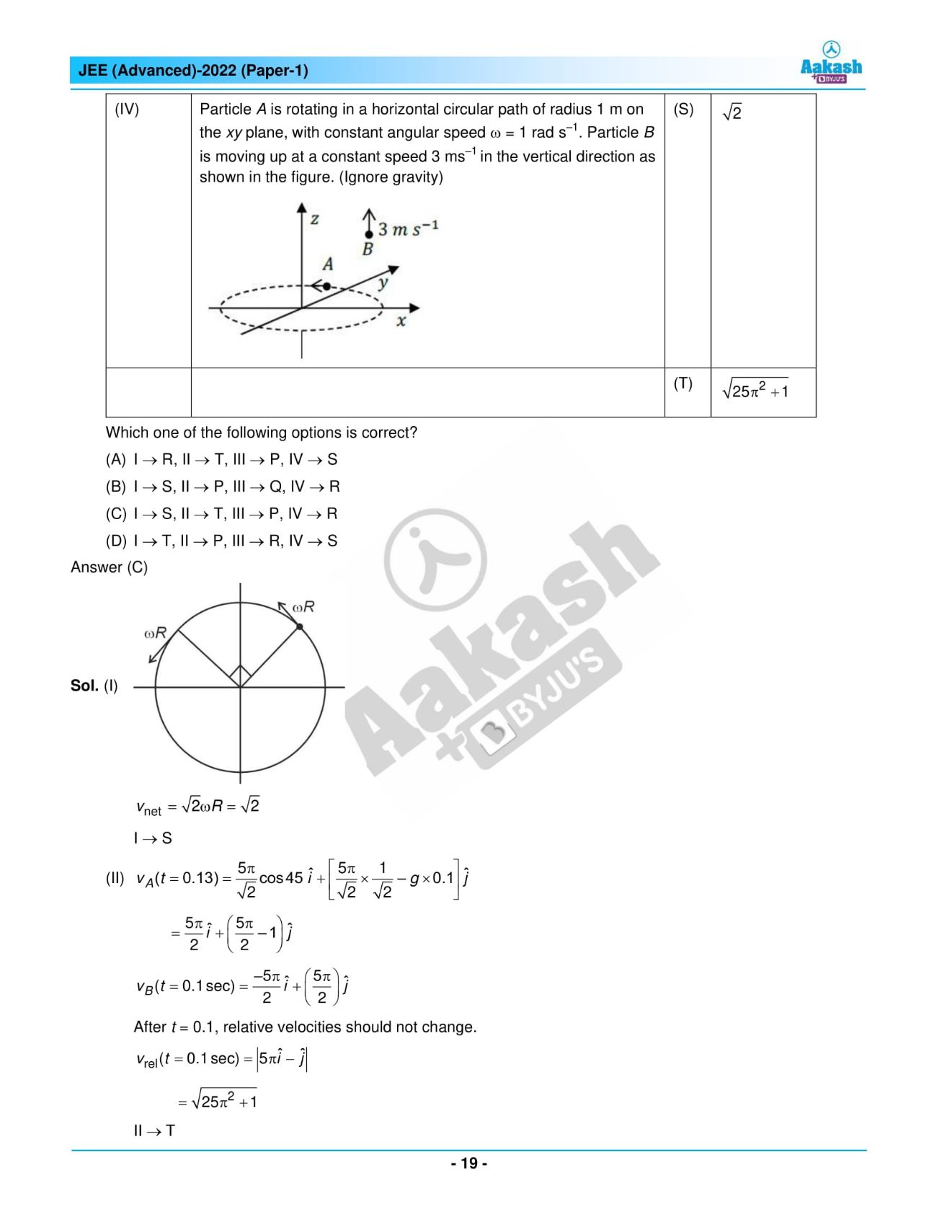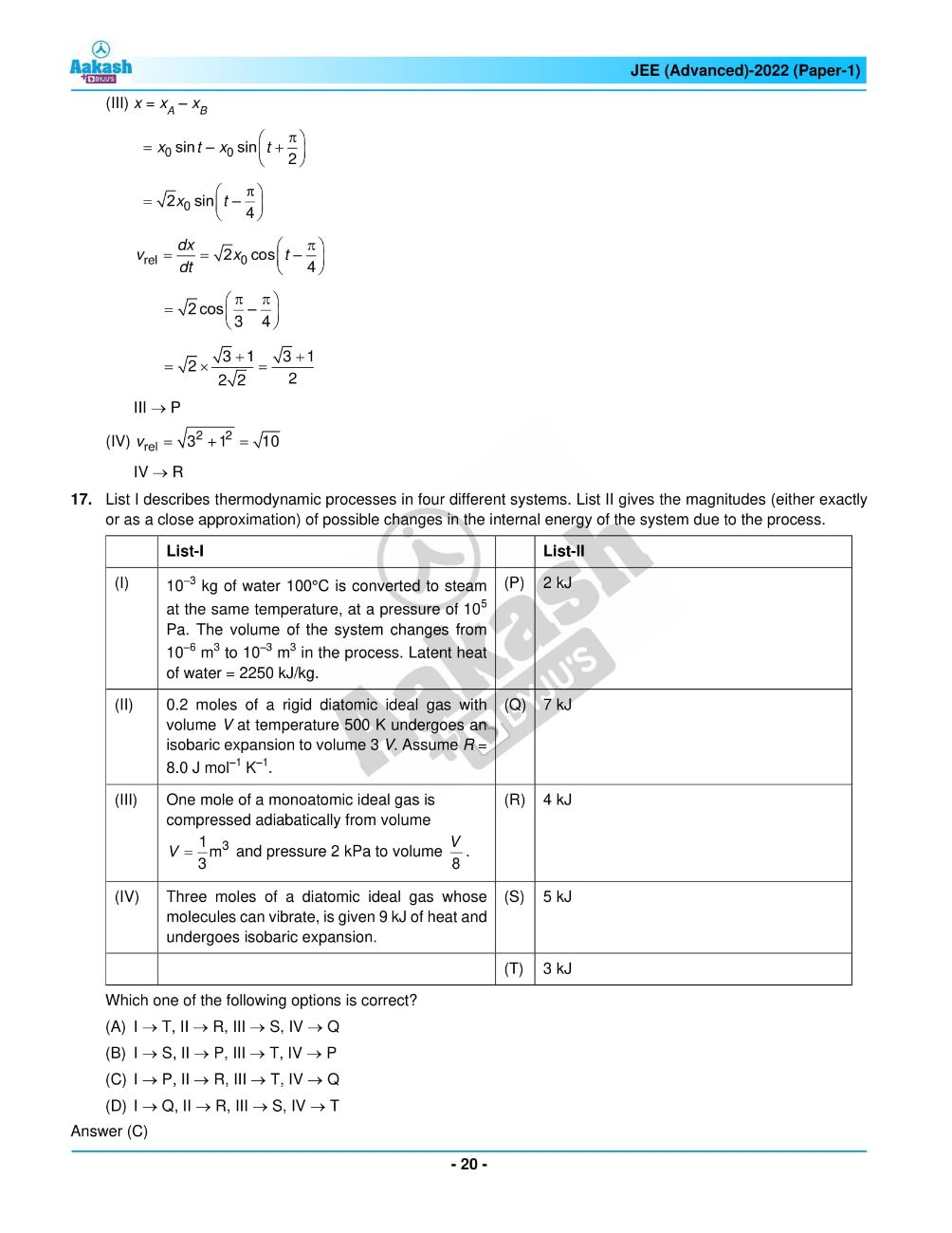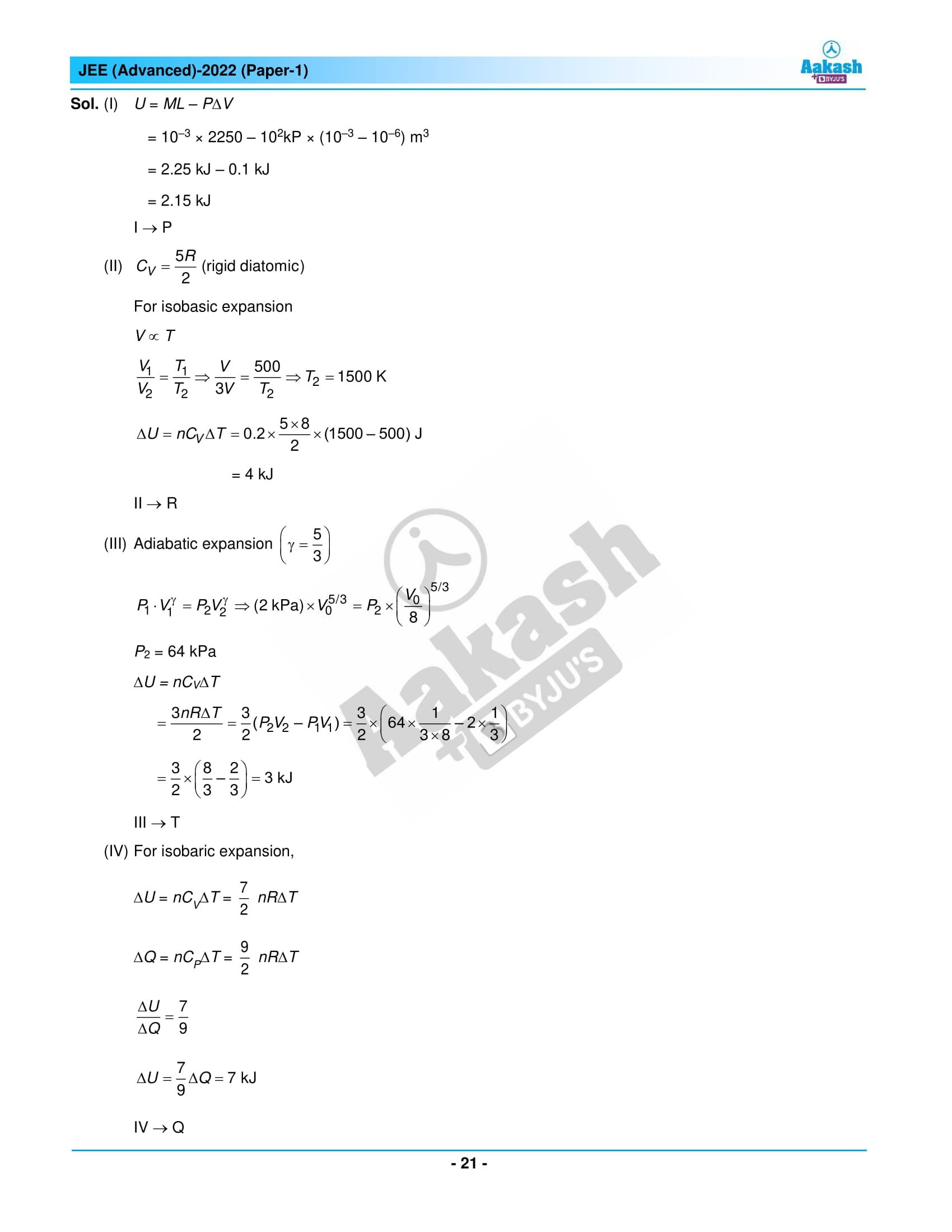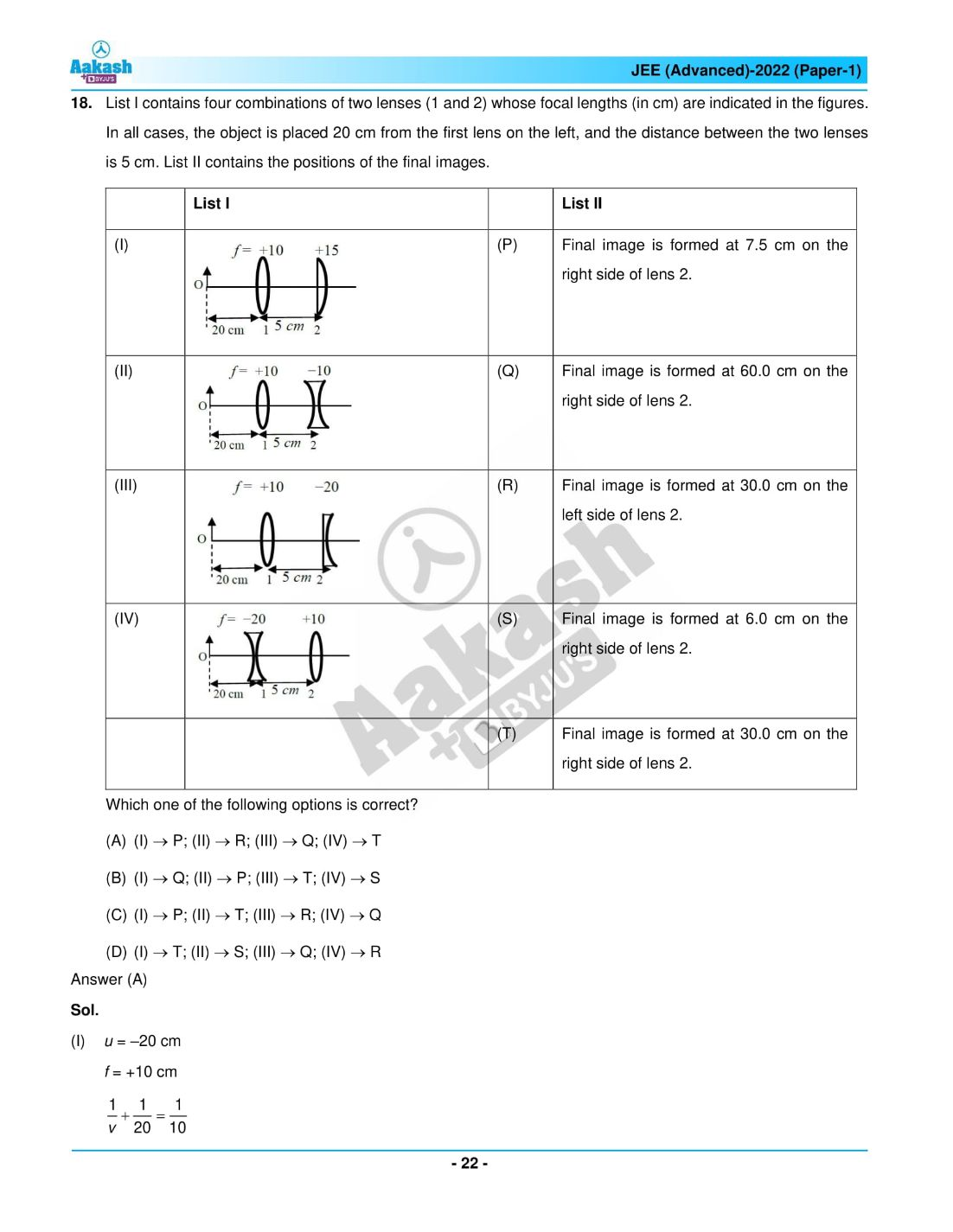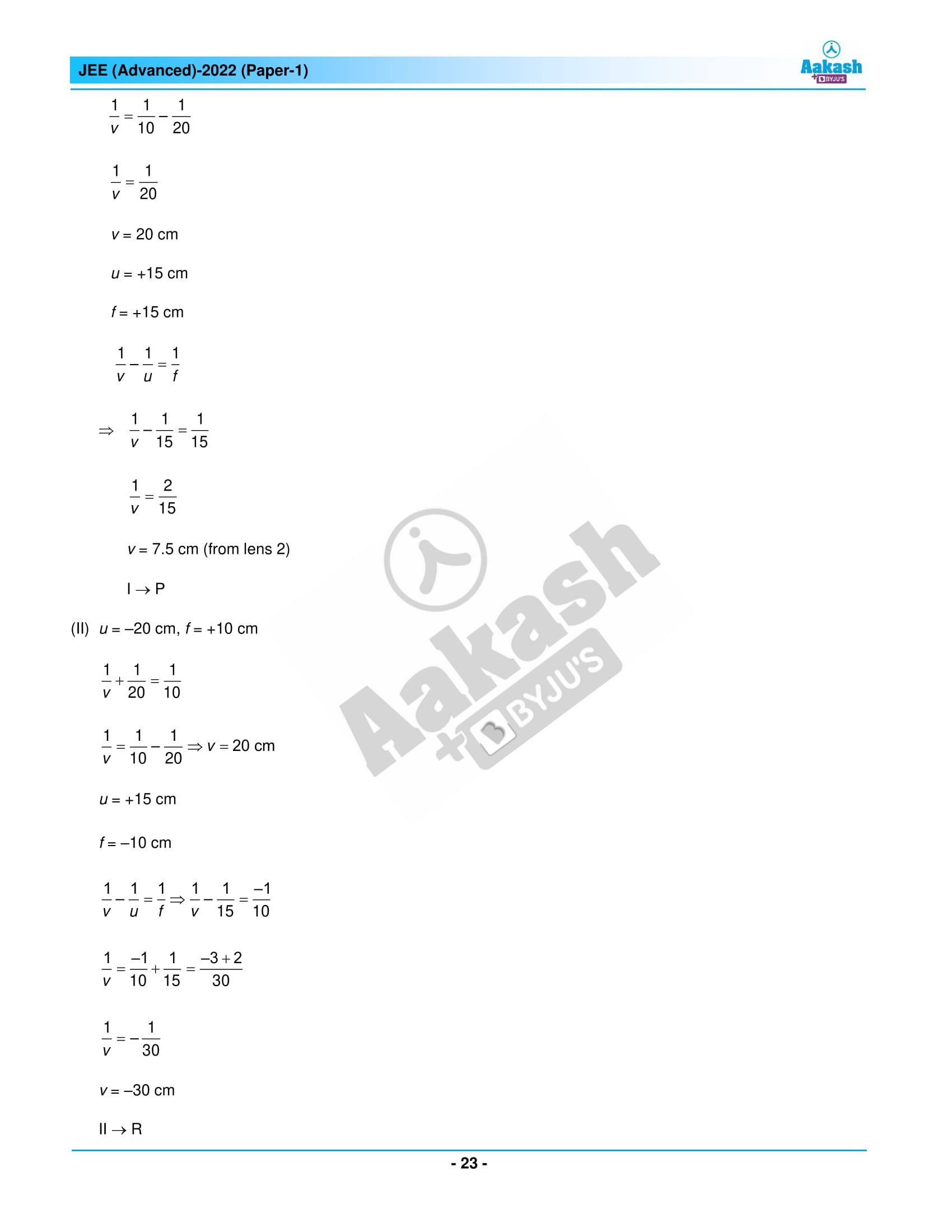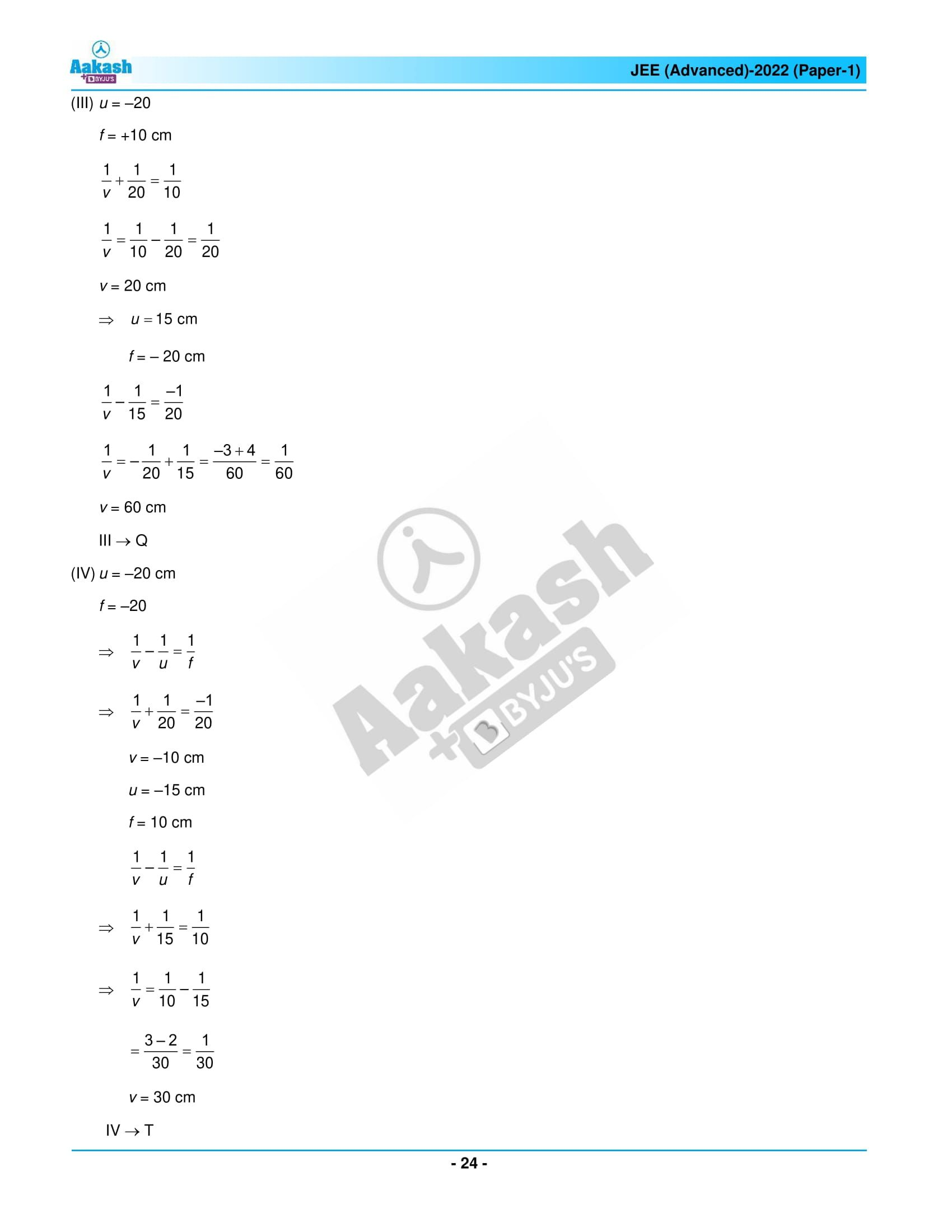## JEE Advanced 2022 Question Paper 1 – Live Paper Analysis and Solutions## Frequently Asked Questions – FAQs

Q1

### How many sections are there in JEE Advanced 2022 Physics Paper 1?

There are three sections in the JEE Advanced 2022 Physics paper 1.
Section 1 – Numerical Value
Section 2 – MCQs more than one correct answer
Section 3 – Matching List Sets (Single Correct Answer)
Q2

### How many easy questions appeared in the JEE Advanced question paper 2022 physics paper 1?

Five easy questions asked from physics in the JEE Advanced question paper 1, 2022.
Q3

### What was the weightage covered for moderately difficult questions in Physics paper 1 of JEE Advanced 2022?

One can score a maximum of 36 marks from the moderately difficult questions in Physics paper 1 of JEE Advanced 2022.
Q4

### Which topics had the difficult questions in the JEE Advanced 2022 Physics paper 1?

Inductance & Inductive Circuits and Thermodynamic Processes had difficult questions in this Physics paper.
Q5

### How do you determine the overall difficulty level of the JEE Advanced 2022 Physics question paper 1?

The overall difficulty level can be rated as 1.83 on a scale of 3, and the difficulty level is the same as the previous year’s paper.